# 1 Tuesday, January 10

References:

• Beauville, “Complex Algebraic Surfaces”
• Huybrechts, “Lectures on K3 Surfaces”
• Gathmann, “Algebraic Geometry” (2002)

K3s are amazing and used in many fields! Named after Kähler, Kodaira, and Kummer. An accomplishment of the early 1900s Italian school of algebraic geometry was classification of complex surfaces (4 real dimensions, admitting holomorphic charts to $${\mathbf{C}}^2$$). These can be studied topologically or using algebraic geometry.

A rough plan:

• Review algebraic varieties:
• Riemann-Roch,
• Curves,
• Divisors,
• Line bundles,
• Picard group,
• The canonical bundle.
• Complex analytic tools:
• The exponential exact sequence,
• Betti numbers,
• Topological Euler characteristic.
• K3s:
• Examples of K3s,
• Enriques-Kodaira classification,
• The intersection form.
• Hodge theory:
• Periods, etc.

Recall the definition of an affine variety, e.g. $$V(x_1, x_2) \subseteq {\mathbf{A}}^2_{/ {k}}$$ the union of the coordinate axes, or the cone $$V(x_1^2-x_2^2-x_3^2) \subseteq {\mathbf{A}}^3_{/ {k}}$$. Alternatively, view them as schemes: $$X = V(f_1,\cdots, f_m) = \operatorname{Spec}k[X]$$ where $$k[X] \mathrel{\vcenter{:}}= k[x_1,\cdots, x_n]/\left\langle{f_1, \cdots, f_m}\right\rangle$$ is the ring of regular functions on $$X$$. The association: for any point $$(a_1,\cdots, a_n) \in k^n$$ one can take the maximal ideals $$\left\langle{x_1-a_1,\cdots, x_n-a_n}\right\rangle$$, this is a bijection by the Nullstellensatz.

An example of why schemes are useful: consider $$V(y)$$ and $$V(y-x^2)$$ in $${\mathbf{A}}^2_{/ {k}}$$. The set-theoretic intersection is $$X\mathrel{\vcenter{:}}= V(y, y-x^2) = \left\{{0}\right\} \in {\mathbf{A}}^2$$, but note that $$k[X] = [x,y]/\left\langle{y, x^2}\right\rangle \neq k = k[x,y]/\left\langle{x,y}\right\rangle$$, so although e.g. $$V(x) = V(x^2)$$ these are distinguished as schemes by remembering the regular functions. A scheme like $$V(x^2)$$ is often drawn as a point with a tangent direction – the scheme remembers not only the values of the $$x_i$$, but also their various partial derivatives.

Recall that varieties carry the Zariski topology: the closed sets are of the form $$V_X(I)$$ for $$I {~\trianglelefteq~}k[X]$$. From a scheme-theoretic perspective, \begin{align*} V(I) \mathrel{\vcenter{:}}=\left\{{\text{prime ideals } p\in X {~\mathrel{\Big\vert}~}p \supseteq I}\right\} .\end{align*}

Consider $$X\mathrel{\vcenter{:}}= V(xy) \subseteq {\mathbf{A}}^2$$, one has $$k[X] = k[x,y]/\left\langle{xy}\right\rangle$$ and $$I=\left\langle{y-x-1}\right\rangle$$ corresponding to the line $$y=x+1$$. What are the closed sets?

Note that $${\mathbf{A}}^1_{/ {{\mathbf{C}}}}$$ with the Zariski topology differs from $${\mathbf{A}}^1_{/ {{\mathbf{C}}}}$$ with the analytic topology. The closed sets are of the form $$V(I)$$, and since $${\mathbf{C}}[x]$$ has GCDs every ideal is principal and $$I = \left\langle{f}\right\rangle \subseteq k[X]$$ for some $$f$$. So closed sets are finite or the entire space, i.e. the cofinite topology. By Serre’s GAGA, miraculously many results and computations are the same in either topology for compact (proper) varieties over $${\mathbf{C}}$$.

Affine varieties/schemes form a category and there is an equivalence $${ {{\mathsf{Aff}}{\mathsf{Sch}}}^{\operatorname{op}}} { \, \xrightarrow{\sim}\, }\mathsf{CRing}$$.

An example of a morphism: \begin{align*} \phi: {\mathbf{A}}^1 &\to {\mathbf{A}}^2 \\ t &\mapsto (t^2, t^3) .\end{align*} This induces a map on regular functions $$\phi^*: {\mathbf{C}}[{\mathbf{A}}^2]\to {\mathbf{C}}[{\mathbf{A}}^1]$$ which is of the form \begin{align*} \phi^*: {\mathbf{C}}[x,y] &\to {\mathbf{C}}[t] \\ x &\mapsto t^2 \\ y&\mapsto t^3 .\end{align*} One could similarly define $$\phi$$ with codomain $$V(y^2-x^3)$$.

What are the regular functions on open sets? Let $$U \subseteq X$$ in the Zariski topology, then regular functions on $$U$$ are ratios $$f/g$$ of polynomials.

Let $$U \mathrel{\vcenter{:}}={\mathbf{A}}^1\setminus\left\{{ 0, 1 }\right\} \subseteq {\mathbf{A}}^1$$, then regular functions include $${1\over x}$$ and $${1\over x-1}$$.

Recall the definition of a sheaf; we’ll write $${\mathcal{O}}_X$$ for the structure sheaf and regard $${\mathcal{O}}_X(U)$$ as the $$k{\hbox{-}}$$algebra of functions on $$U$$, satisfying the sheaf axioms of existence and uniqueness of gluing.

Write $${\mathcal{O}}_{\mathbf{C}}$$ for the sheaf of regular functions, then e.g. $${\mathcal{O}}_{\mathbf{C}}(\left\{{a_1,\cdots, a_n}\right\}^c) = {\mathbf{C}}[x] { \left[ {{1\over x-a_1}, \cdots, {1\over x-a_n}} \right] }$$, and more generally $${\mathcal{O}}_X(V(f)^c) = {\mathbf{C}}[x] { \left[ {1\over f} \right] }$$. We’ll sometimes distinguish $${\mathcal{O}}_{\mathbf{C}}^{\text{hol}}$$ which is defined on $$X^{\mathrm{an}}$$ instead (in the Euclidean topology), which is a priori different as a ringed space. Later we’ll use this in the exponential exact sequence \begin{align*} 2\pi i \underline{{\mathbf{Z}}} \to {\mathcal{O}}&\xrightarrow{\exp} {\mathcal{O}}^{\times}\\ f&\mapsto e^f .\end{align*}

Schemes are useful in number theory: consider $$X \mathrel{\vcenter{:}}=\operatorname{Spec}{\mathbf{Z}}$$, then $${\mathcal{O}}_X(X) = {\mathbf{Z}}$$. There is a point $$p$$ for every prime, and a generic point $$0$$. Note that e.g. $$20\in {\mathcal{O}}_X(X)$$ can be regarded as a function on $$\operatorname{Spec}{\mathbf{Z}}$$, and $$V(20) \mathrel{\vcenter{:}}=\left\{{p {~\mathrel{\Big\vert}~}p\supseteq\left\langle{20}\right\rangle}\right\}$$. It contains $$2$$ and $$5$$, but contains 2 more! So one might draw its “graph” in the following way: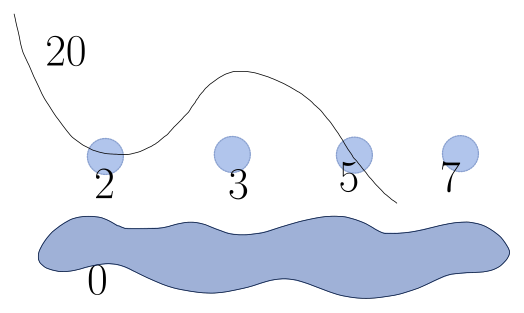Moreover one has $${\mathcal{O}}_{\operatorname{Spec}{\mathbf{Z}}}(\left\{{2,3}\right\}^c) = \left\{{f/g {~\mathrel{\Big\vert}~}f,g\in {\mathbf{Z}}, g = 2^a3^b}\right\}$$.

We can formulate manifolds and varieties in terms of transition functions: for $$U, V \subseteq X$$ and charts $$\phi_U, \phi_V: X\to M$$ for $$M$$ some model space like $${\mathbf{A}}^n$$ or $${\mathbf{R}}^n$$, we can require $$t_{UV} = \phi_V \circ \phi_U^{-1}\mathrel{\Big|}_{\phi_U(U \cap V)}$$ be continuous, smooth, holomorphic, etc. For schemes, the gluing will be by regular maps, e.g. $${\mathbf{P}}^1 = {\mathbf{A}}_1 \amalg_{t\mapsto s = {1\over t} } {\mathbf{A}}_1$$ where $$t,s$$ are the coordinates on each factor.

# 2 Thursday, January 12

Recall the definition of $${\mathbf{P}}^n_{/ {k}}$$ as a variety: lines in $${\mathbf{A}}^{n+1}_{/ {k}}$$ passing through the origin. In the classical topology, it is compact since it can be realized as a quotient $$S^{2n+1}/S^1$$. One can also cover it by affine charts $$U_0 = \operatorname{Spec}{\mathbf{C}} { \left[ {{x_1\over x_0}, {x_2\over x_0}} \right] }$$ and $$U_2, U_3$$ defined similarly, using that $${\left[ {x_0:\cdots x_k \cdots : x_n} \right]} = {\left[ {{x_0\over x_k}:\cdots 1 : \cdots {x_n\over x_k}} \right]}$$ on $$\left\{{x_k\neq 0}\right\}$$. Recall that $${\mathcal{O}}_{{\mathbf{P}}^n}(U) = \left\{{f\in {\mathcal{O}}_{U_i}(U \cap U_i) {~\mathrel{\Big\vert}~}{ \left.{{f}} \right|_{{U \cap U_i}} } = { \left.{{f}} \right|_{{U \cap U_j}} } }\right\}$$.

$${\mathcal{O}}_{{\mathbf{P}}^1}({\mathbf{P}}^1) = \left\{{ (f_0, f_1) \in k[s] \times k[t] {~\mathrel{\Big\vert}~}f_0(s) = f_1(s^{-1}) \text{ on } {\mathbf{A}}^1\setminus\left\{{0}\right\}}\right\}$$ using that $$t=s^{-1}$$ on the overlap. This equals $$k[s] \cap k[s^{-1}] = k$$, so the only global regular functions are constant.

Considering $${\mathbf{P}}^1_{/ {{\mathbf{C}}}}$$ in the analytic (classical) topology, \begin{align*} {\mathcal{O}}_{{\mathbf{P}}^1_{/ {{\mathbf{C}}}}}^\text{hol}({\mathbf{P}}^1_{/ {{\mathbf{C}}}}) = \left\{{f: {\mathbf{P}}^1_{/ {{\mathbf{C}}}}\to {\mathbf{C}}\text{ holomorphic}}\right\} = {\mathbf{C}} .\end{align*}

Since $${\mathbf{P}}^1_{/ {{\mathbf{C}}}}$$ is compact, $$f$$ achieves a maximum value $$m$$ at some point $$p$$, so write $$f(p) = m$$. Letting $$U\ni p$$ be a closed disk containing $$p$$, then $$f$$ has a maximum on $$U$$. By the maximum modulus principle, $${ \left.{{f}} \right|_{{U}} }$$ is constant, and so $$f$$ is constant.

Call $$k[x_0, \cdots, x_{n}]$$ the projective coordinate ring of $${\mathbf{P}}^n_{/ {k}}$$. Recall that $$f \in k[x_0, \cdots, x_{n}]$$ is not a regular function on $${\mathbf{P}}^n$$, but if $$f$$ is homogeneous then $$V(f)$$ is well-defined since $$f(\lambda x_0, \cdots, \lambda x_n) = 0 \iff \lambda^n f(x_0, \cdots, x_n) = 0$$.

Consider $$V(s^2-st) \subseteq {\mathbf{P}}^1_{/ {k}}$$, then $$s(s-t)$$ vanishes on $${\left[ {0: 1} \right]}$$ and $${\left[ {1:1} \right]}$$.

Consider $$V(x^3+y^3+z^3) \subseteq {\mathbf{P}}^2_{/ {{\mathbf{C}}}}$$ – topologically this is $$S^1\times S^1$$ and defines an elliptic curve over $${\mathbf{C}}$$.

$$V(x_0^4 + x_1^4 + x_2^4 + x_3^4) \subseteq {\mathbf{P}}^3_{/ {{\mathbf{C}}}}$$ is a K3 surface.

More generally, $$V(f_1,\cdots, f_m) \subseteq {\mathbf{P}}^n_{/ {k}}$$ with $$f_i$$ homogeneous of degrees $$d_i$$ is a projective scheme, where we use the scheme structure to distinguish e.g. $$V(s^2-st)$$ and $$V(s^3-s^2t)$$.

Some recollections:

• The definition of irreducibility: $$X$$ is reducible if $$X = A\cup B$$ for $$A, B$$ proper nontrivial closed sets.
• E.g. $$V(xy) = V(x) \cup V(y)$$ is not irreducible in $${\mathbf{A}}^2$$.
• Dimension is defined in terms of lengths of chains of closed irreducible subsets.
• $$X$$ is irreducible iff $$k[X] \mathrel{\vcenter{:}}= k[x_1, \cdots, x_{n}]/I(X)$$ is a domain iff $$I(X)$$ is prime
• Krull’s PID theorem, used to show $$\dim R/\left\langle{f}\right\rangle = \dim R - 1$$ if $$f$$ is not a zero divisor
• To see why this is, consider $$R = {\mathbf{C}}[x,y]$$, then $$\dim R/\left\langle{xy}\right\rangle = 1 = \dim {\mathbf{C}}[x] = \dim R/\left\langle{x}\right\rangle$$.
• $$\operatorname{Spec}R$$ is reduced iff $$R$$ has no nilpotents
• E.g. $$V(x^2) = \operatorname{Spec}k[x]/\left\langle{x^2}\right\rangle$$ is not reduced, since $$x$$ is nilpotent ($$x^2=0$$ but $$x\neq 0$$).
• $$X \in {\mathsf{Sch}}$$ is reduced iff $${\mathcal{O}}_X(U)$$ has no nilpotents, so every regular function $$f$$ satisfies $$f^n\neq 0$$ for every $$n$$.
• $$\operatorname{Spec}R$$ is quasicompact.
• E.g. $${\mathbf{A}}^1$$ in the Zariski topology is the cofinite topology, so if $${\mathcal{U}}\rightrightarrows{\mathbf{A}}^1$$ the $$U_1$$ covers all but finitely many points $$p_k$$ and each $$p_k$$ is in some $$U_k$$.
• Open sets are big: they are essentially the whole space, minus lower dimensional things.
• Completeness replaces compactness, where $$X$$ is (universally) complete iff for all $$Y$$, the projection $$X\times Y\to Y$$ is a closed map.
• $${\mathbf{A}}^1$$ is not complete: take $$Y\mathrel{\vcenter{:}}={\mathbf{A}}^1$$, then $$V(xy-1) \mapsto {\mathbf{A}}^1\setminus\left\{{0}\right\}$$ is a closed set mapping to an open set.
• Producing varieties that aren’t manifolds: $$V(xy) \subseteq {\mathbf{A}}^2_{/ {{\mathbf{C}}}}$$ is singular at the origin and has no local chart to $${\mathbf{C}}$$ there.
• $$V(f) \subseteq {\mathbf{R}}^n$$ is a manifold when $$0$$ is a regular value of $$f$$, so $$df: T_p {\mathbf{R}}^n\to T_0 {\mathbf{R}}$$ is surjective at all $$p\in V(f)$$.
• Can be formulated as $$Jf \mathrel{\vcenter{:}}=\qty{{\frac{\partial f_i}{\partial x_j}\,}}$$ has maximal rank everywhere.

# 3 Tuesday, January 17

Recall $$X = V(f_1,\cdots, f_m) = \operatorname{Spec}R \subseteq {\mathbf{A}}^n_{/ {k}}$$ where $$R\mathrel{\vcenter{:}}={\mathbf{C}}[x_1,\cdots, x_n]/\left\langle{f_1,\cdots, f_m}\right\rangle$$ is smooth if $$\operatorname{Jac}\left\{{f_i}\right\} = \qty{{\frac{\partial f_i}{\partial x_j}\,}}$$ has maximal rank $$r = \operatorname{codim}_{{\mathbf{A}}^n} X$$ at all points $$x\in X$$, and we’ll give a more intrinsic notion of smoothness which does not depend on the choice of equations $$\left\{{f_i}\right\}$$. Over $$k={\mathbf{C}}$$, if $$X$$ is smooth it is a complex manifold.

For $$X\mathrel{\vcenter{:}}= V(x^2 + y^2 + 1)$$, note $$\nabla f = {\left[ {2x, 2y} \right]}$$ has rank 1 everywhere except $$0$$, but since $$0\not\in X$$, in fact $$X$$ is smooth.

Recall that $$\Omega^1{R/k} \mathrel{\vcenter{:}}=\bigoplus R\,dr/I$$ where \begin{align*} I = \left\langle{ d(rs) = rds + sdr, d(cr) = cdr, d(r+s) = dr + ds {~\mathrel{\Big\vert}~}r,s\in R, c\in k}\right\rangle .\end{align*} Note that $$\Omega^1_{R/{\mathbf{C}}}\in {}_{R}{\mathsf{Mod}}$$, while $$\Omega^1_{X/{\mathbf{C}}}\in {}_{{\mathcal{O}}_X}{\mathsf{Mod}}$$ is a sheaf.

Example: for $$R = {\mathbf{C}}[x,y]/\left\langle{x^2+y^2+1}\right\rangle$$, we have $$\Omega^1_{R/{\mathbf{C}}} = Rdx + Rdy/I$$. Noting $$x^2+y^2+1=0$$ in $$R$$, we have \begin{align*} 0 = d(x^2+y^2+1) = 2xdx + 2y dy .\end{align*}

$$X$$ is smooth iff the rank of $$\Omega^1_{X/{\mathbf{C}}}$$ at $$p$$ is $$\dim X$$ for every $$p\in X$$. For $$X$$ a variety, point $$p\in X$$ correspond to $${\mathfrak{m}}_p \in \operatorname{mSpec}R$$ and $$\Omega^{1}_{R/{\mathbf{C}}}/{\mathfrak{m}}_x \in \operatorname{mod}{R/{\mathfrak{m}}_p}$$, so we take \begin{align*} \operatorname{rank}_p \Omega^1_{X/{\mathbf{C}}} \mathrel{\vcenter{:}}=\dim_{R/{\mathfrak{m}}_p} (\Omega^1_{R/{\mathbf{C}}}/k) \end{align*} where $$R/{\mathfrak{m}}_p \cong k$$ is a fixed field via the map $$f\mapsto f(p)$$.

The previous example is still smooth: we have \begin{align*} \Omega^1_{R/{\mathbf{C}}}/{\mathfrak{m}}_p = {{\mathbf{C}}dx \oplus {\mathbf{C}}dy \over x(p)dx + y(p) dy} \end{align*} which has $${\mathbf{C}}{\hbox{-}}$$dimension 1 if we don’t have $$x(p) = y(p) = 0$$. This exactly recovers the Jacobi criterion.

An $${\mathcal{O}}_X{\hbox{-}}$$module is a sheaf $${\mathcal{F}}$$ on $$X$$ where

• $${\mathcal{F}}(U) \in {}_{{\mathcal{O}}_X(U)}{\mathsf{Mod}}$$, so the sections are modules over regular functions, and
• $${\mathcal{F}}(U) \xrightarrow{\mathop{\mathrm{Res}}_{UV}} {\mathcal{F}}(V)$$ is compatible with the module structure and $${\mathcal{O}}_X(U) \xrightarrow{\mathop{\mathrm{Res}}_{UV}} {\mathcal{O}}_X(V)$$, so $$\mathop{\mathrm{Res}}_{UV}(f.s) = \mathop{\mathrm{Res}}_{UV}(f) . \mathop{\mathrm{Res}}_{UV}(s)$$ for $$f\in {\mathcal{O}}_X(U)$$ and $$s\in {\mathcal{F}}(U)$$.

$$\Omega^{1}_{X/{\mathbf{C}}}\in {}_{{\mathcal{O}}_X}{\mathsf{Mod}}$$, where the sections are 1-forms on open sets, as is $${\mathcal{O}}_X$$ itself. An example: $$\Omega^1_{{\mathbf{A}}^1\setminus\left\{{0}\right\}} = {\mathbf{C}}[x,x^{-1}]dx$$.

Let $$X = {\mathbf{A}}^1_{/ {{\mathbf{C}}}}$$, then let $${\mathcal{O}}_p$$ be the skyscraper sheaf at $$p$$. This can be made into an $${\mathcal{O}}_X{\hbox{-}}$$module in the following way: for $$f\in {\mathcal{O}}_X(U), s\in {\mathcal{O}}_p$$, define $$f.s = f(p) s$$. How to visualize: think of $${\mathcal{O}}_X$$ as a trivial bundle.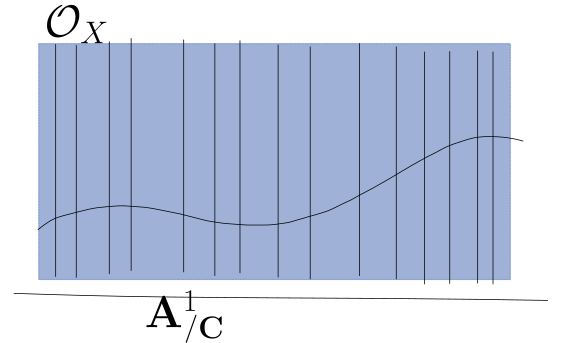Compare to the skyscraper sheaf: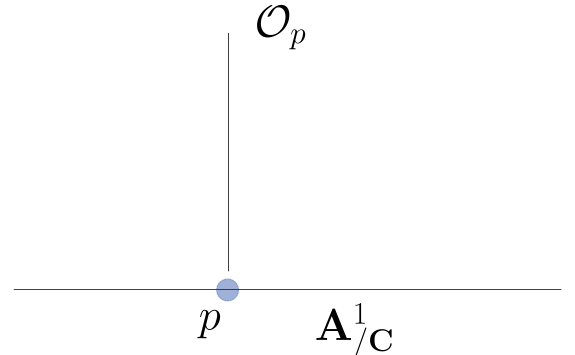If $${\mathcal{F}}, {\mathcal{G}}\in {}_{{\mathcal{O}}_X}{\mathsf{Mod}}$$ then $$\phi: {\mathcal{F}}\to {\mathcal{G}}$$ is a morphism iff it is a morphism of sheaves, so a collection $$\phi(U): {\mathcal{F}}(U) \to {\mathcal{G}}(U)$$, which are compatible with the module actions.

There is a morphism $${\mathcal{O}}_X \to {\mathcal{O}}_p$$ of sheaves determined by \begin{align*} \phi(U): {\mathcal{O}}_X(U) &\to {\mathcal{O}}_p \\ f &\mapsto f(p) ,\end{align*} which is a morphism in $${}_{{\mathcal{O}}_X}{\mathsf{Mod}}$$ since $$(g\cdot f)(p) = g(p)\cdot f(p)$$ is defined by pointwise multiplication.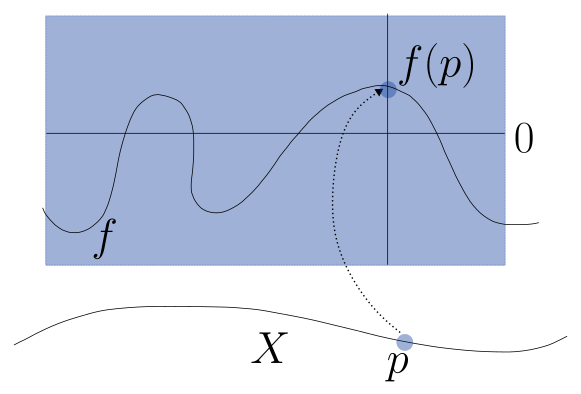Recall that the presheaf $$\ker \phi$$ is a sheaf, and here $$\ker \phi(U) = \left\{{f\in {\mathcal{O}}_X(U) {~\mathrel{\Big\vert}~}f(p) = 0}\right\}$$ is the ideal sheaf of $$p$$, $$I_p$$, so we get a SES \begin{align*} I_p \hookrightarrow{\mathcal{O}}_{{\mathbf{A}}^1_{/ {{\mathbf{C}}}}} \twoheadrightarrow{\mathcal{O}}_p .\end{align*}

For $$V \subseteq W$$ a subvariety, define \begin{align*} I_V(U) = \left\{{f\in {\mathcal{O}}_W(U) {~\mathrel{\Big\vert}~}{ \left.{{f}} \right|_{{V \cap U}} } = 0}\right\} .\end{align*}

Let $$X = {\mathbf{P}}^1_{/ {{\mathbf{C}}}}$$, then recall $${\mathcal{O}}_X(X) = {\mathbf{C}}$$ in this case. Letting $$p\neq q\in X$$, there is a map \begin{align*} {\mathcal{O}}_X &\to {\mathcal{O}}_p \oplus {\mathcal{O}}_q \\ f &\mapsto f(p) \oplus f(q) .\end{align*} This is a surjection of sheaves, despite not being surjective on global sections: $${\mathcal{O}}_X(X) = {\mathbf{C}}$$, while $$({\mathcal{O}}_p \oplus {\mathcal{O}}_q)(X) = {\mathbf{C}}\oplus {\mathbf{C}}$$. However, this is an open cover $${\mathcal{U}}\rightrightarrows X$$ where this is a surjection on sections: take $$U_1 \mathrel{\vcenter{:}}= X\setminus\left\{{ p }\right\}$$ and $$U_2\mathrel{\vcenter{:}}= X\setminus\left\{{ q }\right\}$$. Here we get a SES \begin{align*} I_{\left\{{p, q}\right\}} \hookrightarrow{\mathcal{O}}_{{\mathbf{P}}^1_{/ {{\mathbf{C}}}}}\twoheadrightarrow{\mathcal{O}}_p \oplus {\mathcal{O}}_q .\end{align*}

For $$X = {\mathbf{A}}^1_{/ {{\mathbf{C}}}}$$, there is an isomorphism \begin{align*} \Omega^1_{X} & { \, \xrightarrow{\sim}\, }{\mathcal{O}}_X \in {}_{{\mathcal{O}}_X}{\mathsf{Mod}}\\ f &\mapsto f\,dx .\end{align*}

For $$X$$ not affine, what is $$\Omega^1_{X}$$? If $$\omega = \sum f_i dx_i$$ in one chart and $$\sum g_i dy_i$$ in another via charts $$\vec x, \vec y$$, how are they related? One needs a notion of pullbacks. We define $$\Omega^1_X(U)$$ to be well-defined 1-forms $$\omega_i \in \Omega^1_X(U \cap U_i)$$ which are compatible on overlaps.

Let $$X = {\mathbf{P}}^1$$, glued from affines $$U_0 = \operatorname{Spec}{\mathbf{C}}[s]$$ and $$U_1 = \operatorname{Spec}{\mathbf{C}}[t]$$ by \begin{align*} t_{01}: U_0 &\to U_1 \\ s &\mapsto t=s^{-1} .\end{align*}

Take $$\omega_i \in \Omega^1_X(U_i)$$, then

• $$\omega_0 = f_0(s)ds \in {\mathbf{C}}[s] ds$$
• $$\omega_1 = f_1(t)dt \in {\mathbf{C}}[t] dt$$

Then the compatibility condition is that \begin{align*} t_{01}^*(\omega_1) = f_1(s^{-1})d(s^{-1}) = f_0(s) ds .\end{align*}

This becomes \begin{align*} - {f_1(s^{-1}) \over s^2}ds &= f_0(s) ds \\ \implies f_1(s^{-1}) &= -s^2 f_0(s)\\ \implies c_0 + c_1 s^{-1}+ c_2 s^{-2} + \cdots + c_k s^{-k} &= d_0 s^2 + d_1 s^3 + \cdots + d_r s^r ,\end{align*} which can only be true if $$f \equiv 0$$. This implies that $$\Omega^1_{X}$$ is a line bundle.

A line bundle on $$X$$ is $${\mathcal{F}}\in {}_{{\mathcal{O}}_X}{\mathsf{Mod}}$$ where $$\exists {\mathcal{U}}\rightrightarrows X$$ where $${ \left.{{{\mathcal{F}}}} \right|_{{U_i}} } = {\mathcal{O}}_{U_i}$$. A vector bundle of rank $$r$$ is such an $${\mathcal{F}}$$ where $${ \left.{{{\mathcal{F}}}} \right|_{{U_i}} } = {\mathcal{O}}_{U_i}{ {}^{ \scriptscriptstyle\oplus^{r} } }$$ for some $$r$$.

$${\mathcal{O}}_p$$ is not a vector bundle, since $${\mathcal{O}}_p(U)\not { \, \xrightarrow{\sim}\, }{\mathcal{O}}_X(U){ {}^{ \scriptscriptstyle\oplus^{r} } }$$ for any $$r$$ or any affine open $$U\ni p$$.

A Weil divisor on $$X$$ is a $${\mathbf{Z}}{\hbox{-}}$$linear combination of irreducible codimension 1 subvarieties. For $$D = \sum n_i p_i$$, its degree is $$\sum n_i$$.

The irreducible codimension 1 subvarieties of $${\mathbf{P}}^1$$ are points, so \begin{align*} \operatorname{WDiv}({\mathbf{P}}^1) = \bigoplus _{p\in {\mathbf{P}}^1} {\mathbf{Z}}[p] .\end{align*} For example, $$\operatorname{WDiv}({\mathbf{P}}^1_{/ {{\mathbf{C}}}})\ni D \mathrel{\vcenter{:}}= 2 - [\pi] + 3[\infty]$$ and $$\deg D = 4$$. Similarly, $$\operatorname{WDiv}({\mathbf{A}}^2)\ni [V(x)] - [V(y)]$$.

Let $$X$$ be irreducible and $$f\in {\mathcal{O}}_X(U)$$ with $$U \subseteq X$$ Zariski open, and define $$\operatorname{div}(f) \mathrel{\vcenter{:}}=\sum n_i [D_i]$$ where $$n_i$$ is the order of zeros/poles of $$f$$ along $$D_i$$.

Let $$x/y^2 \in {\mathcal{O}}_{{\mathbf{A}}^2}(V(y)^c)$$, then $$\operatorname{div}(x/y^2) = [V(x)] - 2[V(y)]$$. Similarly, $$f\mathrel{\vcenter{:}}={s^2-t^2\over st}\in {\mathcal{O}}_{{\mathbf{P}}^1}({\mathbf{P}}^1\setminus\left\{{ 0, \infty }\right\})$$ has divisor $$\operatorname{div}(f) =  + [-1] -  - [\infty]$$.

# 4 Thursday, January 19 (Divisors)

Recall that $$\exp$$ is surjective as a map of sheaves. On open contractible subsets $$U \subseteq {\mathbf{C}}$$, for any $$g\in {\mathcal{O}}_{\text{hol}}^{\times}(U)$$ there is an $$f\mathrel{\vcenter{:}}=\log(g)$$, but $$z\mapsto \log(z)\not\in {\mathcal{O}}_{\text{hol}}({\mathbf{C}}^{\times})$$. Thus surjections of sheaves need not induce surjections on global sections, the failure is measured by sheaf cohomology.

Define the principal Weil divisors as \begin{align*} \mathop{\mathrm{Prin}}\mathrm{W}Div = \left\{{\operatorname{div}(f) {~\mathrel{\Big\vert}~}f\in K(X)}\right\} ,\end{align*} divisors of nonzero rational functions. Here $$\operatorname{div}(f) = \sum n_Y [Y]$$ where $$n_Y$$ is the order of vanishing/poles along $$Y$$. We then define the (Weil) divisor class group as \begin{align*} \mathrm{W} \operatorname{Cl}(X) \mathrel{\vcenter{:}}=\operatorname{WDiv}(X) / \mathop{\mathrm{Prin}}\operatorname{Div}(X) .\end{align*}

On $${\mathbf{P}}^1$$, $$\operatorname{div}\qty{s^2-t^2\over st} =  + [-1] -  - [\infty]$$, regarding $$\infty = s/t$$.

$$\operatorname{Cl}({\mathbf{A}}^1) = 0$$ since $$\sum n_p [p] = \operatorname{div}f$$ where $$f = \prod (x-p)^{n_p}$$.

There is an isomorphism \begin{align*} \deg: \mathrm{W} \operatorname{Cl}({\mathbf{P}}^1) & { \, \xrightarrow{\sim}\, }{\mathbf{Z}}\\ \sum n_p [p] &\mapsto \sum n_p .\end{align*} E.g. considering $$\operatorname{div}\qty{}$$ $$ + [-1] -  = [\infty]$$ in $$\operatorname{Cl}({\mathbf{P}}^1)$$.

Consider $$\operatorname{Cl}(\operatorname{Spec}{\mathbf{Z}})$$: principal divisors are primes, so $$\operatorname{WDiv}(\operatorname{Spec}{\mathbf{Z}}) = \left\{{\sum_{p\text{ prime}} n_p [p] }\right\}$$. Rational functions on $$\operatorname{Spec}{\mathbf{Z}}$$ are identified with $${\mathbf{Q}}$$, and if $$r = \prod p_i^{n_i}\in {\mathbf{Q}}$$ then $$\operatorname{div}(r) = \sum n_i [p_i]$$, so $$\operatorname{Cl}(\operatorname{Spec}{\mathbf{Z}}) = 0$$ since every $$\sum_{p \text{ prime}} n_p [p]$$ is the divisor of some $$r\in {\mathbf{Z}}\subseteq {\mathbf{Q}}$$.

For $$X = \operatorname{Spec}R$$ for $$R\mathrel{\vcenter{:}}={\mathbf{Z}} { \left[ {\sqrt{-5}} \right] }$$, we have $$\operatorname{WDiv}(X) = \sum_{p\neq 0 \text{ prime ideals}} n_p [p]$$, and the rational functions on $$X$$ are $${\mathbf{Q}}(\sqrt{-5})$$. Since $$R\in \mathbb{D}$$, there is unique factorization of (fractional) ideals, so writing $$(r) = \prod p_i^{n_i}$$ we have $$\operatorname{div}r = \sum n_i [p_i]$$. However, $$R$$ is not a UFD, considering $$2\cdot 3 = (1 + \sqrt{-5})(1 - \sqrt{-5})$$.

Consider $$r = 1\cdot [p]$$ for $$p = (2, 1 + \sqrt{-5})$$? Ask.

A Cartier divisor is a collection of rational functions $$f_i$$ on $$U_i$$ such that $$\operatorname{div}(f_i) = \operatorname{div}(f_j)$$ on $$U_{ij}$$. These form a group $$\operatorname{CDiv}(X)$$, and there is a corresponding class group $$\mathrm{Ca} \operatorname{Cl}(X) \mathrel{\vcenter{:}}=\operatorname{CDiv}(X) / \mathop{\mathrm{Prin}}\operatorname{CDiv}(X)$$.

Write $${\mathbf{P}}^1 = U_0 \cup U_1$$ and consider

• $$f_0 = s$$ on $$U_0 = \operatorname{Spec}{\mathbf{C}}[s]$$
• $$f_1 = 1$$ on $$U_1 = \operatorname{Spec}{\mathbf{C}}[t]$$

Note $$\operatorname{div}f_0 = $$ on $$U_0$$ and $$\operatorname{div}f_1 = 0$$ on $$U_1$$, but $${ \left.{{f_0}} \right|_{{U_{01}}} }$$ has no poles or zeros and thus $$\operatorname{div}{ \left.{{f_0}} \right|_{{U_{01}}} }= 0 = \operatorname{div}{ \left.{{f_1}} \right|_{{U_{01}}} }$$.

If $$X$$ is smooth then $$\operatorname{WDiv}(X) = \operatorname{CDiv}(X)$$. Note all Weil divisors are Cartier: consider $$X = V(xy-z^2) \subseteq {\mathbf{A}}^3$$, which is a circular cone. Note that $$V(z)$$ is a union of two lines along the edge of the cone. Consider $$D = V_X(z, y)$$, an irreducible codimension 1 subvariety, so $$D\in \operatorname{WDiv}(X)$$. This is locally principal away from the origin, since one can slice by the plane $$z=0$$. Suppose $$f(x,y,z)$$ cuts out $$D$$ at $$0$$, then write $$f = c_0 + c_1x + c_2y + c_3z + \cdots$$. Since $$f(0) = 0$$ we have $$c_0 = 0$$, and the remaining terms always cut out two lines. On the other hand, $$2D$$ is Cartier and principal, since the tangent plane along the cone $$V_X(y) = V_X(y, z^2)$$ cuts out a doubled line.

Recall that line bundles are $${\mathcal{L}}\in {}_{{\mathcal{O}}_X}{\mathsf{Mod}}$$ locally isomorphic to $${\mathcal{O}}_{U_i}$$. Given $$D\in\operatorname{CDiv}(X)$$ with Cartier data $$\left\{{(f_i, U_i)}\right\}$$ with $$f_i$$ rational on $$U_i$$ and $$\operatorname{div}(f_i) = \operatorname{div}(f_j)$$ on overlaps. Define $${\mathcal{O}}_X(D)\in\operatorname{Pic}(X)$$ to be the sheaf whose sections over $$U$$ are $$\left\{{ (s_i) \in {\mathcal{O}}_X(U \cap U_i) s_i f_i = s_j f_j }\right\}$$, so the sections are related by $$s_j = {f_i\over f_j} s_i$$ on $$U_{ij}$$. Write $$t_{ij} = {f_j\over f_i}\in {\mathcal{O}}_X(U_i \cap U_j)$$ for the transition functions.

Write $${\mathbf{P}}^1 = U_0 \cup U_1$$ and $$D = \left\{{(s, U_0), (1, U_1)}\right\} \in \operatorname{CDiv}({\mathbf{P}}^1)$$, and consider $${\mathcal{O}}_{{\mathbf{P}}^1}(D)$$. This is given by $$\left\{{p\in k[s], q\in k[t] {~\mathrel{\Big\vert}~}p = sq}\right\}$$. Writing $$t=s^{-1}$$, we have $$p(s) = sq(s^{-1})$$, so if $$q=1$$ then $$p=s$$ and if $$q(t) = t$$ then $$p=1$$. One can check $${\mathcal{O}}_{{\mathbf{P}}^1}(D) = {\mathbf{C}}\left\langle{s, 1}\right\rangle \oplus {\mathbf{C}}\left\langle{1, t}\right\rangle$$.

Show that if $$D = \left\{{(s^k, U_0), (1, U_1)}\right\}$$ then $${\mathcal{O}}_{{\mathbf{P}}^1}(D) = {\mathcal{O}}_{{\mathbf{P}}^1}(k)$$, whose global sections are homogeneous degree $$k$$ polynomials on $${\mathbf{P}}^1$$.

# 5 Tuesday, January 24

Recall that $$\operatorname{WDiv}(X) = \mathrm{Ca}\operatorname{Div}(X)$$ if $$X$$ is smooth or if $$\operatorname{codim}X_{\mathrm{sing}}\geq 3$$, and for any $$D\in \operatorname{CDiv}(X)$$, \begin{align*} {\mathcal{O}}_X(D)(U) \mathrel{\vcenter{:}}=\left\{{f\in k(U) {~\mathrel{\Big\vert}~}\operatorname{div}f + D \geq 0}\right\}\in \operatorname{Pic}(X) \end{align*} is a line bundle.

Let $$D = V(xyz) \subseteq {\mathbf{P}}^2$$, then $$D$$ is 3 copies of $${\mathbf{P}}^1$$ linked in a triangle. Consider $$f\in {\mathcal{O}}_{{\mathbf{P}}^2}(D)({\mathbf{P}}^2)$$, so $$\operatorname{div}f = -L_1 -L_2 -L_3 + \sum n_p P$$ for some $$n_p \geq 0$$. Since $$f\in k({\mathbf{P}}^2)\implies f = a/b$$ with $$a,b$$ homogeneous polynomials of the same degree, one example is $$f(x,y,z) = a(x,y,z)/xyz$$ with $$a$$ homogeneous of degree 3. Thus $${\mathsf{H}}^0({\mathcal{O}}_{{\mathbf{P}}^2}(D)) \cong k[x,y,z]^{3, { \mathrm{homog} }}$$.

If $$D \equiv D'\in \operatorname{Cl}(X)$$, so $$D-D'= \operatorname{div}h$$ for some $$h$$, then $${\mathcal{O}}_X(D)\cong {\mathcal{O}}_X(D')$$ as sheaves.

One needs a map $${\mathcal{O}}_X(D)(U) \to {\mathcal{O}}_X(D')(U)$$ for every open $$U \subseteq X$$, so take the map \begin{align*} {\mathcal{O}}_X(D')(U) &\to {\mathcal{O}}_X(D)(U) \\ f' &\mapsto f\mathrel{\vcenter{:}}= f'\cdot h^{-1} .\end{align*} Since $$\operatorname{div}(f' h^{-1}) = \operatorname{div}(f') - \operatorname{div}(h)$$, we have \begin{align*} \operatorname{div}f' + D' \geq 0 \iff \operatorname{div}f' + D' + \operatorname{div}h \geq \operatorname{div}h \iff \operatorname{div}f + D \geq 0 .\end{align*}

Note that if $$D\geq 0$$ then $${\mathcal{O}}_X(D)(X) \ni 1$$, the constant function, and this is a global section so $$H^0({\mathcal{O}}_X(D)) > 0$$.

Any irreducible codimension 1 $$D \subseteq {\mathbf{P}}^n$$ is of the form $$V(f)$$ for a single function $$f$$, which follows from the fact that any height 1 prime in $$k[x_0, \cdots, x_{n}]$$ is principal. Thus $$\operatorname{Div}({\mathbf{P}}^n) = \left\{{\displaystyle\sum_{f\in k[x_0,\cdots, x_n]^{ { \mathrm{homog} }, {\mathrm{irr}}} } n_f [V(f)] }\right\}$$, and if $$D = \sum n_f [V(f)]$$ and $$D' = \sum n_{f'}[V(f')]$$, then $$D\equiv D' \iff \sum n_f \deg f = \sum n_{f'} \deg f'$$, noting $$D-D' = \operatorname{div}\qty{\prod f^{n_f} \over \prod (f')^{n_{f'}} }$$ which is a rational function on $${\mathbf{P}}^n$$. So $$\operatorname{Cl}({\mathbf{P}}^n)\underset{\deg}{ { \, \xrightarrow{\sim}\, }} {\mathbf{Z}}$$ where $$\sum n_f [V(f)] \mapsto \sum n_f \deg f$$.

$$\operatorname{Pic}(X)$$ is the group of line bundles on $$X$$ up to isomorphism, with group structure given by the following: for $$L_1, L_2\in \operatorname{Pic}(X)$$, define \begin{align*} (L_1\otimes L_2)(U) \mathrel{\vcenter{:}}= L_1(U)\otimes_{{\mathcal{O}}_X(U)} L_2(U) .\end{align*} Alternatively, the transition functions on the tensor product are products of transition functions: \begin{align*} t_{UV}^{L_1\otimes L_2} = t_{UV}^{L_1}\cdot t_{UV}^{L_2} .\end{align*} The identity element is $${\mathcal{O}}_X$$, since $$L_1(U) \otimes_{{\mathcal{O}}_X(U)} {\mathcal{O}}_X(U) = L_1(U)$$. Inverses are given by $$L^{-1}\mathrel{\vcenter{:}}=\mathop{\mathcal{H}\! \mathit{om}}(L, {\mathcal{O}}_X)$$, so $$L^{-1}(U) = \mathop{\mathrm{Hom}}_{{\mathcal{O}}_X(U)}(L(U), {\mathcal{O}}_X(U))$$ on small enough open sets, and the transition functions are given by \begin{align*} t_{UV}^{L^{-1}} = (t_{UV}^{L})^{-1} .\end{align*} It can be checked that if $$L$$ satisfies the cocycle condition iff $$L^{-1}$$ does, and similarly for $$L_1\otimes L_2$$.

If $$X$$ is smooth then $$\operatorname{Pic}(X)\cong \operatorname{Cl}(X)$$ via $$D\rightleftharpoons{\mathcal{O}}_X(D)$$.

This uses that $${\mathcal{O}}_X(D)\cong{\mathcal{O}}_X(D')\iff D\equiv D'$$, the interesting part is to show surjectivity. Let $$L\in \operatorname{Pic}(X)$$, then $${ \left.{{L}} \right|_{{U}} } = {\mathcal{O}}_U$$ for some $$U$$, and we can consider $$1\in {\mathcal{O}}_U(U) \cong L(U)$$. In any other trivialization, $${ \left.{{L}} \right|_{{V}} } \cong {\mathcal{O}}_V$$ and there is a transition function $$t_{UV} \in k(V)$$. Since $$1\in {\mathcal{O}}_U(U)$$, we have $$\operatorname{div}(1) = D$$ where the LHS is regarded as a rational section of $$L$$, and $$L \cong {\mathcal{O}}_X(D)$$.

A rational section of $$L$$ is a section of $$L\otimes k(X)$$.

This allows for a section $$s\in H^0(L)$$ to have poles, and $$L\cong {\mathcal{O}}(\operatorname{div}(s))$$ for any section $$s$$ of $$L$$. If $$s, s'$$ are rational sections, then $$s/s'$$ is a rational function. Concretely, if $$s = \left\{{s_u \in k(U) {~\mathrel{\Big\vert}~}t_{UV} s_U = s_V}\right\}$$ and $$s' = \left\{{s'_U \in k(U) {~\mathrel{\Big\vert}~}t_{UV} s'_U = s'_V}\right\}$$. Then $$s/s' = \left\{{s_U \over s_U' = s_V/s_V'}\right\} \in k(X)$$, so $$\operatorname{div}(s) = \operatorname{div}(s')$$.

The degree of any principal divisor on a curve is zero.

More interesting examples come from elliptic curves. Write $$X = {\mathbf{C}}/ \Lambda$$ where $$\Lambda = {\mathbf{Z}}\oplus {\mathbf{Z}}\tau$$ with $$\tau \in {\mathbb{H}}$$ This yields a complex manifold, since the transition functions are translations and thus holomorphic. We can write $$\operatorname{Div}(X)\ni D = \sum n_p [p]$$ where $$p\in X$$ are points. A meromorphic function is a rational function $$f: X\dashrightarrow{\mathbf{C}}$$ which extends to a holomorphic map $$f:X\to {\mathbf{CP}}^1$$ by mapping poles to $$\infty$$ – note that this extension only works because $$X$$ is complex dimension 1, and does not work in higher dimensions. This pulls back to $$\tilde f: {\mathbf{C}}\to {\mathbf{P}}^1$$ which satisfies $$\tilde f(z+ \lambda) = \tilde f(z)$$ for all $$\lambda\in \Lambda$$. The Weierstrass $$\wp{\hbox{-}}$$function is defined by \begin{align*} \wp(z) \mathrel{\vcenter{:}}={1\over z^2} + \sum_{\lambda\in \Lambda\setminus\left\{{0}\right\}}\qty{ {1\over (z - \lambda)^2 } - {1\over \lambda^2} } ,\end{align*} which averages over the lattice and is thus periodic. Note \begin{align*} {1\over (z- \lambda)^2} - {1\over \lambda^2} = { \lambda^2 - (z- \lambda)^2 \over (z- \lambda)^2 \lambda^2} .\end{align*} where the denominator is $$\geq C {\left\lvert { \lambda} \right\rvert}^4$$ for $${\left\lvert {z} \right\rvert} \gg 1$$ and the numerator is $$\leq c {\left\lvert { \lambda} \right\rvert}$$ for $${\left\lvert {z} \right\rvert} \gg 1$$, so \begin{align*} \sum_{ \lambda \in \Lambda\setminus\left\{{0}\right\}} C{\left\lvert {\lambda} \right\rvert}^{-3} \leq C \int_{{\mathbf{R}}^2} {\left\lvert {\lambda} \right\rvert}^{-3} = C \iint r^{-3} r\,dr\,d\theta \end{align*} which converges. So the extra constant $${1\over \lambda^2}$$ is necessary to make the series converge. Since translating by $$\lambda\in \Lambda$$ rearranges the series, $$\wp(z)$$ is a well-defined rational function on $$X$$ with a double pole at every $$z\in \Lambda$$, corresponding to $$0\in {\mathbf{C}}/ \Lambda$$. So $$\operatorname{div}\wp(z) = -2 $$, and it induces $$X\xrightarrow{\pi} {\mathbf{P}}^1$$ where we have $$\deg \pi = \deg \pi^{-1}(0) = \deg \pi^{-1}(\infty)$$.

Let $$f\in {\mathbf{C}}(X)$$ be meromorphic and let $$\operatorname{div}f = \sum n_p [p]$$.

1. $$\sum n_p = 0$$
2. $$\sum n_p [p] \equiv 0 \operatorname{mod}\Lambda$$

Let $$f\in {\mathbf{C}}(X)$$ with some zeros and poles.

Take the following contour: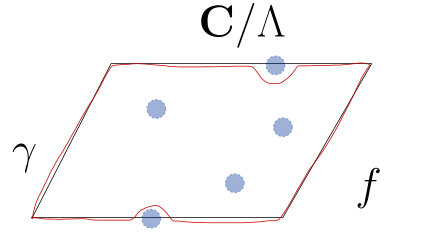Note that $$\int_\gamma \operatorname{dLog}(f) = \int_\gamma {f'(z) \over f(z)} \,dz= \sum_{p \text{ inside}} n_p$$ by the residue theorem. Recall that in local coordinates $$w$$, if $$f(w) = cw^{-k} + \cdots$$ then $$\operatorname{dLog}(f) = -k {dw \over w} + h(w)$$ where $$h$$ is holomorphic. However, by periodicity, the edge integrals cancel and $$\int_{C_1 + C_2} \operatorname{dLog}(f) = \sum_{p\text{ edge}} -n_p$$, forcing $$\sum n_p = 0$$.

Now considering $$\int_\gamma z\operatorname{dLog}(f) = \sum_{p \text{ inside}} n_p p$$ and $$\mathop{\mathrm{Res}}_p z\operatorname{dLog}(f) = n_p \cdot z(p)$$. On the other hand, $$-\sum_{p \text{ edge}} n_p p+ \int_{A\setminus A'} z\operatorname{dLog}(f)$$.

# 6 Thursday, February 02

If $$f:C\to D$$ is a map of smooth complete curves then \begin{align*} 2g(C) - 2 = \deg(f)\cdot\qty{ 2g(D) - 2 } + \deg R(f) \end{align*} where $$R(f) = \sum_{p \in C} (e_p - 1)[p]$$ is the ramification divisor.

Take \begin{align*} {\mathbf{C}}&\to {\mathbf{C}}\\ z &\mapsto z^{4} \end{align*} which is a 4-fold cover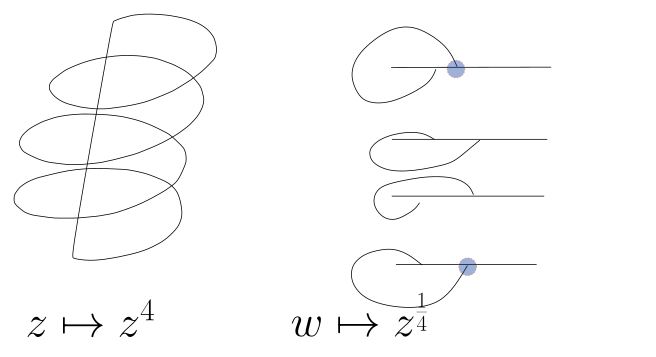If $$f: C\to {\mathbf{P}}^1$$ is degree 2 then $$f=a/b$$ where $$a,b\in H^0(C; K_C)$$, and Riemann-Hurwitz gives $$\deg R(f) = 6$$. If $${\left[ {s: t} \right]}$$ are homogeneous coordinates on $${\mathbf{P}}^1$$, then one can take an equation of the form $$z^2 = f_6(s, t)$$ for $$f_6(s,t) = \prod_{i=1}^6 (a_i s - b_i t)$$ is a homogeneous degree 6 polynomial, so $$f\in H^0({\mathbf{P}}^1;{\mathcal{O}}_{{\mathbf{P}}^1}(6))$$ and $$z\in { \operatorname{Tot} }{\mathcal{O}}_{{\mathbf{P}}^1}(-3)$$:Describe why $$z\not\in { \operatorname{Tot} }{\mathcal{O}}_{{\mathbf{P}}^1}(3)$$ instead.

Recall that the normalization of a ring $$R$$ is the integral closure of $$R$$ in $$\operatorname{ff}(R)$$. Compute the normalization of $$y^2=x^3$$ using the algebraic definition.

An example of normalization:Integrally closed and 1-dimensional implies smooth.

Recall the definition of the Čech cochain complex and compute $${\check{H}}(S^1; {\mathcal{F}})$$ using an open cover of two sets.

# 7 Tuesday, February 07

Last time: Čech cochains defined as $$C_{{\mathcal{U}}}^p(X; {\mathcal{F}}) \mathrel{\vcenter{:}}=\bigoplus_{i_0 < \cdots < i_p } {\mathcal{F}}(U_{i_0,\cdots, i_p})$$ with homology defined as $${\check{H}}^*(X;{\mathcal{F}}) \mathrel{\vcenter{:}}=\colim_{{\mathcal{U}}} H^*(C_{\mathcal{U}}^*(X; {\mathcal{F}}))$$, and a SES of sheaves yields a LES in homology. Recall $$\operatorname{Cl}(X) { \, \xrightarrow{\sim}\, }\operatorname{Pic}(X)$$ in our setting via $$D\mapsto {\mathcal{O}}_X(D)$$ which sends $$U$$ to $$\left\{{f\in k(U) {~\mathrel{\Big\vert}~}\operatorname{div}f + D \geq 0}\right\}$$.

\begin{align*} \operatorname{Pic}(X) & { \, \xrightarrow{\sim}\, } H^1(X; {\mathcal{O}}_X^{\times}) \\ L &\mapsto [(t_{UV})] .\end{align*}

Write $$C_{{\mathcal{U}}}^1(X; {\mathcal{O}}_X^{\times}) = \left\{{ ( t_{UV} ) \in {\mathcal{O}}^{\times}( U \cap V) {~\mathrel{\Big\vert}~}U,V \in {\mathcal{U}}}\right\}$$ for some open cover $${\mathcal{U}}\rightrightarrows X$$, and $$Z_{{\mathcal{U}}}^1(X; {\mathcal{O}}_X^{\times}) = \left\{{(t_{UV}) \in {\mathcal{O}}^{\times}(U \cap V)}\right\}$$ with boundary $${{\partial}}^1(t_{UV}) = (t_{VW} t_{UW}^{-1}t_{UV})$$. Note that $$t_{UW} = t_{UV} t_{VW}$$.

A line bundle $$L \xrightarrow{\pi} X$$ has a trivialization, and we can refine $${\mathcal{U}}$$ to a cover that trivializes $$L$$, so $${ \left.{{L}} \right|_{{U}} } \cong {\mathcal{O}}_U$$ as sheaves for each $$U$$. We have $$\pi^{-1}(U) { \, \xrightarrow{\sim}\, }U\times {\mathbf{C}}$$, and $$h_V\circ h_U^{-1}: (U \cap V) \times {\mathbf{C}}\to (U \cap V)\times {\mathbf{C}}$$ is multiplication by $$t_{UV}(p)$$ on the fiber over $$p \in U \cap V$$. These satisfy $$t_{UV} t_{VW} = t_{UW}$$, so any $$L$$ defines an element of $$Z^1(X; {\mathcal{O}}_X^{\times})$$ by sending $$L$$ to its transition function.

Conversely, given $$(t_{UV})$$, one can attempt to glue these to form a line bundle, but which collections define the same bundle? Given two line bundles, refine their trivializing covers so that they coincide. Then any two trivializations $$L_U \xrightarrow{h_U, h_{U}'} {\mathcal{O}}_U$$ differ by an element $$f_U \in {\mathcal{O}}^{\times}(U)$$:On overlaps, we have $$t_{UV}\mapsto f_U^{-1}t_{UV}$$ and $$t_{VU} \mapsto f_U t_{VU}$$, so at the level of tuples, $$(t_{UV}) \mapsto (t_{UV}) \cdot {\partial}^0 (f_U)$$ and thus $$(t_{UV})$$ is uniquely defined up to $${\partial}^0(C_{\mathcal{U}}^0(X; {\mathcal{O}}_X^{\times}))$$.

This works for higher rank vector bundles: one has $$t_{VW} t_{UV} = t_{UW}$$ in $$\mathop{\mathrm{Hol}}(U \cap V \cap W, \operatorname{GL}_n({\mathbf{C}}))$$, however for $$n\geq 2$$ this is a nonabelian group and order matters. In this case we get e.g. $${\partial}^1(t_{UV}) = ( t_{UV} t{UW}^{-1}t_{VW} )$$. One has \begin{align*} \left\{{\text{Vector bundles on } X}\right\} { \, \xrightarrow{\sim}\, }H^1(X; \operatorname{GL}_n({\mathcal{O}})) \end{align*} where $$\operatorname{GL}_n({\mathcal{O}})(U) = \left\{{\text{holomorphic functions } U\to \operatorname{GL}_n({\mathbf{C}})}\right\}$$.

Recall the exponential SES; taking the LES yields $$c_1: \operatorname{Pic}(X) \to H^2(X; {\mathbf{Z}})$$. For $$X$$ a smooth curve, $$c_1 = \deg$$. For $$D\in \operatorname{Div}(X)$$, one can define the fundamental class in $$X$$ by taking the fundamental class $$[D]\in H_{\dim_{\mathbf{R}}D}(D; {\mathbf{Z}}) { \, \xrightarrow{\sim}\, }H_{\dim_{\mathbf{R}}D} (X; {\mathbf{Z}}) \underset{\mathrm{PD}}{ { \, \xrightarrow{\sim}\, }} H^2(X; {\mathbf{Z}})$$.

Why is $$c_1 = \deg$$ true? Consider $${\mathcal{L}}= {\mathcal{O}}_C(p)$$ for $$p\in C$$ a point on a curve. One can take the point bundle construction: let $$U\ni p$$ be a neighborhood of $$p$$ and $$V$$ the complement of a smaller neighborhood of $$p$$, so $$U \cap V$$ is an annulus. For $$z: U\to {\mathbf{C}}$$ a local coordinate, one can form a Cartier divisor $$\left\{{(U, z), (V, 1)}\right\}$$ with transition function $$t_{UV} = 1/z$$. Note that $$H^0({\mathcal{O}}_C(p)) \ni s = (s_U = z, s_V = 1)$$ has a section which vanishes precisely at $$p$$.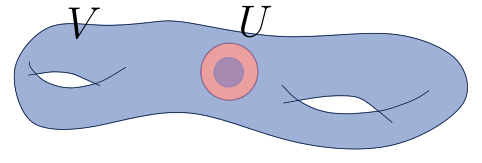Refine the open cover to split $$U$$ into two open subsets, then \begin{align*} c_1({\mathcal{O}}_C(p)) = ((z^{-1})_{U_1V}, (z^{-1})_{U_2V}, (1)_{U_1 U_2} ) \in Z^1(C; {\mathcal{O}}^{\times}) .\end{align*} Lifting to $$C^1(C; {\mathcal{O}})$$ using that exponential surjects on sheaves yields $$(-\log(z), -\log(z), 0) \in C^1(C; {\mathcal{O}})$$. Taking its boundary yields \begin{align*} {\partial}^1( (-\log z)_{U_1 V}, (-\log z)_{U_2 V}, (0)_{U_1 U_2} ) = ( (-\log z)_{U_2 V} + (\log z)_{U_i V} )_{U_1 U_2 V} \end{align*} which is $$0$$ on the top component of $$U_1 \cap U_2 \cap V$$ and $$2\pi i$$ on the bottom. This is an element of $$\underline{2\pi i {\mathbf{Z}}}( U_1 \cap U_2 \cap V) \in Z^2(C; \underline{2\pi i {\mathbf{Z}}}) \to H^2(X; \underline{{\mathbf{Z}}} )$$. Thus $$c_1 ({\mathcal{O}}_C(p)) = [p]$$ is the fundamental class of $$p$$.

\begin{align*} {\operatorname{NS}}(X) \mathrel{\vcenter{:}}=\operatorname{im}c_1, \quad \operatorname{Pic}^0(X) \mathrel{\vcenter{:}}=\ker c_1 .\end{align*}

For $$X = E$$ an elliptic curve, $$\operatorname{Pic}X = E\times {\mathbf{Z}}$$ where $$D \mapsto (D, \deg D)$$. Thus $${\operatorname{NS}}(E) = {\mathbf{Z}}$$ and $$E = \operatorname{Pic}^0(E) = \left\{{[p] -  {~\mathrel{\Big\vert}~}p\in E}\right\}$$. Note that $$\operatorname{Pic}^0(X) \cong \operatorname{Jac}(X)$$ in this case.

If $$X$$ is smooth projective, global holomorphic functions are constant, so part of the LES breaks into an exact piece: \begin{align*} H^0({\mathbf{Z}}) = {\mathbf{Z}}^n \hookrightarrow H^0({\mathcal{O}}) = {\mathbf{C}}^n \twoheadrightarrow H^0({\mathcal{O}}^{\times}) = ({\mathbf{C}}^{\times})^n \qquad n = {\sharp}\pi_0 X .\end{align*} Thus $$\operatorname{Pic}^0(X) = H^1(X; {\mathcal{O}})/ H^1(X; {\mathbf{Z}})$$, and Hodge theory shows $$\operatorname{rank}H^1(X; {\mathbf{Z}}) = 2\dim_{\mathbf{C}}H^1(X; {\mathcal{O}})$$ and the image of $$H^1(X;{\mathbf{Z}})\to H^1(X; {\mathcal{O}})$$ is discrete. This yields $${\mathbf{Z}}^{2r} \hookrightarrow{\mathbf{C}}^r$$ with image $$\Lambda$$ a lattice and $$\operatorname{Pic}^0 X \cong {\mathbf{C}}^r/\Lambda$$. In particular, for $$C$$ a smooth genus $$g$$ curve, $$\operatorname{Pic}^0 X \cong {\mathbf{C}}^g/ \Lambda$$. Note that $$H^1(C; {\mathbf{Z}})$$ carries the intersection pairing, which induces a symplectic form and thus a polarization.

# 8 Thursday, February 09

Last time: $$\operatorname{Pic}(X) \cong H^1(X; {\mathcal{O}}_X^{\times})$$ and \begin{align*} c_1: \operatorname{Pic}(X) &\to H^2(X; {\mathbf{Z}}) \\ {\mathcal{O}}(D) &\mapsto [D] \end{align*} with $$\operatorname{im}c_1 = {\operatorname{NS}}(X)$$ and $$\ker c_1 = \operatorname{Pic}^0(X) \cong {\mathbf{C}}^g/ \Lambda$$ where $$H^1(X; {\mathcal{O}}) \cong {\mathbf{C}}^g$$. Today: consider the cohomology of vector bundles on a complex manifold $$X$$.

A smooth $$(p, q){\hbox{-}}$$form is locally of the form \begin{align*} \sum_{ {\left\lvert {I} \right\rvert} = p, {\left\lvert {J} \right\rvert} = q} a_{I, \overline{J}} \,dz_{i_1}\wedge\cdots \,dz_{i_p} \wedge \,d\overline{z}_{j_1}\wedge \cdots \wedge \,d\overline{z}_{j_q} .\end{align*} Let $$A^{p, q}$$ be the sheaf of smooth $$(p, q){\hbox{-}}$$forms

Some examples:

• $$A^{0, 1}({\mathbf{C}}) \ni \omega \mathrel{\vcenter{:}}= z\overline{z} \,d\overline{z}$$.
• $$A^{1, 0}({\mathbf{C}}^{\times}) \ni \alpha \mathrel{\vcenter{:}}=\log{\left\lvert {z} \right\rvert} \,dz$$
• $$A^{1,1}({\mathbf{C}}^2)\ni \alpha \mathrel{\vcenter{:}}= e^{z_1}\,dz_1 \wedge \,d\overline{z}_2 + \overline{z}_2 \,d\overline{z}_1 \wedge \,dz_2$$.
• An example of differentiation: $$d(e^{z_2} \,dz_1 + \,d\overline{z}_1 \,dz_2) = e^{z_2} \,dz_2\wedge \,dz_1 + \,d\overline{z}_1 \wedge \,dz_2$$.

Let $$\Omega^{p}$$ be the holomorphic $$(p, 0)$$ forms, noting that differentiation $$d$$ on smooth forms is not a map of $$C^\infty(X, {\mathbf{C}}){\hbox{-}}$$modules since $$d(f\alpha) = fd(\alpha) + df\wedge \alpha$$ for $$f \in C^\infty(U, {\mathbf{C}})$$ and $$\alpha A^k(U)$$. There is a decomposition \begin{align*} A^k(U) = \bigoplus _{p+q=k} A^{p, q}(U) ,\end{align*} which leads to a decomposition of sheaves. Define $${\partial}= \pi_{p+1, q}(d)$$ and $${ \overline{{\partial}}}: \pi_{p, q+1}(d)$$, this yields a complex \begin{align*} 0 \to {\mathcal{O}}_X \to A^{0,0} \xrightarrow{{ \overline{{\partial}}}} A^{0, 1} \xrightarrow{{ \overline{{\partial}}}} A^{0, 2} \to \cdots \to A^{0, \dim X} \to 0 ,\end{align*} which is an exact sequence of sheaves. Noting that $$d^2 = 0$$, one has

• $${\partial}^2 = 0$$,
• $${ \overline{{\partial}}}^2 = 0$$,
• $${\partial}{ \overline{{\partial}}}+ { \overline{{\partial}}}{\partial}= 0$$.

See the Poincaré $${ \overline{{\partial}}}$$ lemma.

More generally, for a vector bundle $$E \in {}_{{\mathcal{O}}_X}{\mathsf{Mod}}$$, note that $${\mathcal{O}}\hookrightarrow A^{0, 0}$$ yields $${\mathcal{O}}\hookrightarrow{\mathbf{C}}^\infty$$, so can form $$E\otimes_{{\mathcal{O}}} C^\infty$$. Locally, $$E\cong {\mathcal{O}}{ {}^{ \scriptscriptstyle\oplus^{r} } }$$ on $$U$$, so one has $$E\otimes A^{0,0} \cong (C^\infty){ {}^{ \scriptscriptstyle\oplus^{r} } }$$ on $$U$$. This yields $$0\to E\hookrightarrow E \otimes A^{0,0}$$, and the claim is that there is a well-defined map $$E\otimes A^{0,0}\to E\otimes A^{0, 1}$$. Locally this is given by $${\left[ {f_1,\cdots, f_r} \right]} \mapsto {\left[ {{ \overline{{\partial}}}f_1,\cdots, { \overline{{\partial}}}f_r} \right]}$$. In a different trivialization, $$s_V = t_{UV}(f_1,\cdots, f_r)$$ where $$t_{UV}$$ is a holomorphic function valued in $$\operatorname{GL}_r({\mathbf{C}})$$. One has $${ \overline{{\partial}}}(t_{UV} \circ (f_1,\cdots, f_r)) = t_{UV} ({ \overline{{\partial}}}f_1, \cdots, { \overline{{\partial}}}f_r)$$ since $${ \overline{{\partial}}}(t_{UV}) = 0$$, noting that in the first expression one is carrying out matrix multiplication.

\begin{align*} 0 \to E \xrightarrow{i} E\otimes A^{0,0} \xrightarrow{{ \overline{{\partial}}}} E\otimes A^{0, 1} \xrightarrow{{ \overline{{\partial}}}} \cdots \xrightarrow{{ \overline{{\partial}}}} E\otimes A^{0, \dim X} \to 0 ,\end{align*} and $$H^*(X; E)$$ can be computed from the homology of this complex.

For any smooth vector bundle $$V\to M$$ over a manifold $$M$$, $${\check{H}}^{i \geq 1}(M; V) = 0$$ since $$M$$ admits partitions of unity. Moreover if $${\mathcal{F}}\hookrightarrow{ {I}_{\scriptscriptstyle \bullet}}$$ with $${ {I}_{\scriptscriptstyle \bullet}}$$ acyclic, so $$H^{i\geq 1}({ {I}_{\scriptscriptstyle \bullet}} ) = 0$$, then $$H^k(X; F)$$ is computed as the homology of $${ {I}_{\scriptscriptstyle \bullet}}$$.

Since $$\Omega^p$$ is a holomorphic vector bundle on $$X$$, this yields the Dolbeault resolution \begin{align*} 0\to \Omega^p \to A^{p, 0} \to A^{p, 1} \to \cdots ,\end{align*} and $$H^{p, q} \mathrel{\vcenter{:}}= H^q(X; \Omega^p)$$ is the homology of this complex. Define $$h^{p, q} \mathrel{\vcenter{:}}=\dim_{\mathbf{C}}H^{p, q}$$ – note that this forms a diamond since for $$p,q\geq \dim X$$ there are no $$p{\hbox{-}}$$forms or $$q{\hbox{-}}$$forms whatsoever.

There is a decomposition \begin{align*} H^k(X; \underline{{\mathbf{C}}}) \cong \bigoplus _{p+q=k} H^{p, q} ,\end{align*} and a symmetry \begin{align*} H^{p, q}(X) \cong \overline{H^{q, p}(X)} .\end{align*}

Note that $$\overline{V}$$ doesn’t necessarily make sense yet for $$V = H^{p, q}(X)$$, since we don’t know that it is a subspace of some real vector space $$W$$ – here we’ll take $$H^k(X; \underline{{\mathbf{C}}}) = H^k(X; \underline{{\mathbf{R}}}) \otimes{\mathbf{C}}$$. E.g. writing $${\mathbf{C}}^2 = {\mathbf{R}}^2 \otimes_{\mathbf{R}}{\mathbf{C}}$$, if $$V = \left\langle{u, v}\right\rangle_{\mathbf{C}}$$ then $$\overline{V} = \left\langle{\overline{u}, \overline{v}}\right\rangle_{\mathbf{C}}$$.

# 9 Tuesday, February 14

\begin{align*} h^0(C, L) - h^0(C, K_C \otimes L^{-1}) = \deg L + 1-g .\end{align*}

Recall:

• $$h^i(X, F) \mathrel{\vcenter{:}}=\dim_k H^i(X; F)$$.
• $$\chi(X, F) \mathrel{\vcenter{:}}=\sum_{i\geq 0}h^i(X, F)$$ for $$F\in {\mathsf{Sh}}(X, {}_{k}{\mathsf{Mod}})$$, provided these numbers are finite.
• $$H^0({\mathbf{A}}^1_{/ {k}}; {\mathcal{O}}) = k[x] t^0$$, and note $$\dim_k k[x] = \infty$$.
• For $$X$$ an irreducible Noetherian topological space with $$\dim X = d$$, $$H^i(X, F) = 0$$ for $$i > d$$.
• For $$X\in \mathop{\mathrm{Proj}}{\mathsf{Var}}_{/ {k}}$$ and $$F$$ a finitely presented $${\mathcal{O}}_X{\hbox{-}}$$module, i.e. there is an exact sequence $${\mathcal{O}}_X{ {}^{ \scriptscriptstyle\oplus^{m} } }\to {\mathcal{O}}_X{ {}^{ \scriptscriptstyle\oplus^{n} } }\twoheadrightarrow F$$, we have $$h^i(X, F) < \infty$$.
• Finitely presented sheaves are coherent. An analytic coherent sheaf is defined in the same way with respect to $${\mathcal{O}}_X^{\mathrm{an}}$$ (the sheaf of holomorphic functions).
• $$h^0(X, K_C\otimes L^{-1}) = h^1(C, L)$$.

Let $$X$$ be a compact complex manifold and let $$E\to X$$ be a holomorphic vector bundle. Then $$H^i(X, E) { \, \xrightarrow{\sim}\, }H^{\dim_{\mathbf{C}}X - i}(X, E {}^{ \vee }\otimes K_X) {}^{ \vee }$$ where $$K_X = \operatorname{det}\Omega_X \mathrel{\vcenter{:}}=\Omega_X^{\dim X}$$.

Regard $$s\in H^i(X, E)$$ as an element in Dolbeault cohomology, \begin{align*} H^i(X, E) \cong { \ker \qty{ E\otimes A^{0, i}(X) \xrightarrow{{ \overline{{\partial}}}} A^{0, i+1}(X) } \over \operatorname{im}\qty{E\otimes A^{0, i-1}(X) \xrightarrow{{ \overline{{\partial}}}} E\otimes A^{0, i}(X) } } .\end{align*} Note that $$K \otimes_{\mathcal{O}}C^\infty = A^{n, 0}$$. Let

• $$t\in H^{n-i}(X, E {}^{ \vee }\otimes K)$$
• $$\tilde s\in E\otimes A^{0, i}(X)$$
• $$\tilde t\in E {}^{ \vee }\otimes K \otimes A^{0, n-i}(X) \cong E {}^{ \vee }\otimes A^{n, n-i}(X)$$.

One can then pair $${\left\langle {\tilde s},~{\tilde t} \right\rangle}\in A^{n, n}(X)$$, and $$\int_X {\left\langle {\tilde s},~{\tilde t} \right\rangle} \in {\mathbf{C}}$$ is a perfect pairing.

Upshot: the LHS in RR is $$\chi(C, L)$$.

The following is an important exact sequence of sheaves: for any $$D\in \operatorname{CDiv}(X)^\mathsf{eff}$$, one has \begin{align*} {\mathcal{O}}_X(-D) \hookrightarrow{\mathcal{O}}_X \twoheadrightarrow{\mathcal{O}}_D \qquad \in {\mathsf{Sh}}(X) \end{align*} where $${\mathcal{O}}_D \mathrel{\vcenter{:}}=\iota_* {\mathcal{O}}_X$$ for $$\iota: D\hookrightarrow X$$ the inclusion.

Note $${\mathcal{O}}_X(D)(U) = \left\{{f\in {\mathcal{O}}_(U) {~\mathrel{\Big\vert}~}\operatorname{div}f \geq D}\right\}$$, so $${\mathcal{O}}_X(D) = I_D$$ is the ideal sheaf of $$D$$. If $$D$$ is cut out by a single function on $$U$$, we have $$I_D(U) = (f) \subset {\mathcal{O}}_X(U)$$. This yields an inclusion $${\mathcal{O}}_X(-D) = I_D \hookrightarrow{\mathcal{O}}_X$$. By definition, the quotient $${\mathcal{O}}_X/I_D$$ are functions defined on $$D$$, at least on affine opens $$U$$. Since exactness of sheaves is local, this check suffices.

In particular, on a curve one has \begin{align*} {\mathcal{O}}_C(-p) \hookrightarrow{\mathcal{O}}_C \twoheadrightarrow{\mathcal{O}}_p \end{align*} where $$p\in C$$ is a point. This can be tensored with any vector bundle $$L$$ to get \begin{align*} L(-p) \mathrel{\vcenter{:}}= L\otimes{\mathcal{O}}_C(-p) \hookrightarrow L \twoheadrightarrow{ \left.{{L}} \right|_{{p}} } ,\end{align*} which is exact since $$L$$ is locally free. For $$s_p\in H^0({\mathcal{O}}_C(p))$$, we have $$V(s_p) = [p]$$ as a divisor: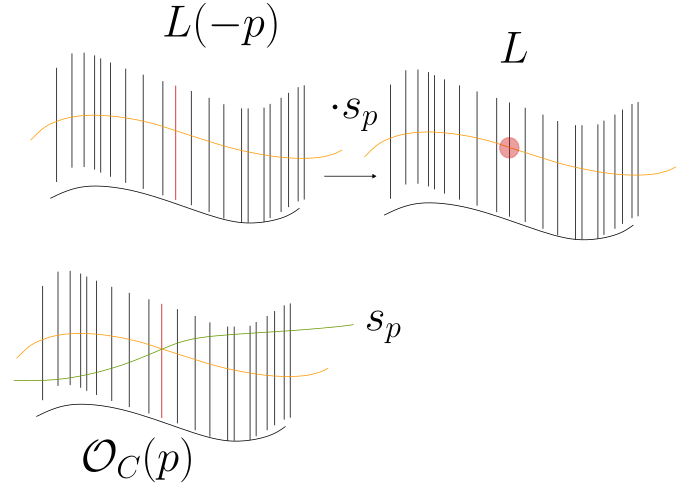For $$F_1\hookrightarrow F_2 \twoheadrightarrow F_3$$, \begin{align*} \chi(F_2) = \chi(F_1) + \chi(F_3) .\end{align*}

Take the LES in cohomology, where $$H^n(F_i) = 0$$ for large enough $$n$$. Now for a LES of vector spaces $$V_1\hookrightarrow V_2\to \cdots \twoheadrightarrow V_n$$, one has $$\sum (-1)^i \dim_k V_i = 0$$.

$${ \left.{{L}} \right|_{{p}} } \cong {\mathcal{O}}_p$$ satisfies $$H^i(C, {\mathcal{O}}_p) = {\mathbf{C}}t^0$$, which is more generally true for a skyscraper sheaf at a point.

Take a fine enough open cover (e.g. an affine cover) so that $$p$$ appears in only one set, and use Čech cohomology. That $${ \left.{{L}} \right|_{{p}} } \cong {\mathcal{O}}_p$$ follows from the fact that this holds on a small enough open $$U$$ and both are identically zero away from $$p$$.

Now use that $$\chi(C, {\mathcal{O}}_p) = 1$$, we then claim that $$\chi(L) = \chi(L(-p)) + 1$$ and thus $$L\cong {\mathcal{O}}_C(\sum n_p [p])$$ and $$\chi(L) = \sum n_p + \chi({\mathcal{O}}_C)$$ by repeatedly applying this fact. Note $$\sum n_p = \deg L$$, so \begin{align*} \chi(L) = \deg L + \chi({\mathcal{O}}_C) .\end{align*} We have $$\chi({\mathcal{O}}_C) = h^0({\mathcal{O}}_C) - h^1({\mathcal{O}}_C) = 1- h^0(K)$$ by Serre duality. Applying the above version of RR to $$K$$ yields $$\chi(K) = \deg K + \chi({\mathcal{O}}) = 2g-2 + \chi({\mathcal{O}})$$. On the other hand, this equals $$h^0(K) - h^0(K {}^{ \vee }\otimes K) = h^0(K) - 1$$. Combining these yields \begin{align*} \chi({\mathcal{O}}) = -(2g-2 + \chi({\mathcal{O}})) \implies 2\chi({\mathcal{O}}) = 2-2g \implies \chi({\mathcal{O}}) = 1-g .\end{align*} Plugging this back into the first equation yields \begin{align*} \chi(L) = \deg L + 1-g .\end{align*}

Note that $$\chi^{\mathsf{Top}}(C) = g$$ was defined as the index of a vector field, and this shows that also $$g = h^1({\mathcal{O}}_X)$$.

An application of Serre duality: the Hodge diamond. Let $$n\mathrel{\vcenter{:}}=\dim_{\mathbf{C}}X$$ and recall $$h^{p, q} \mathrel{\vcenter{:}}=\dim_{\mathbf{C}}H^q(X, \Omega^p_X)$$. By duality, $$h^{p, q} = \dim_{\mathbf{C}}H^{n-q}(X, (\Omega_X^p) {}^{ \vee }\otimes\Omega^n)$$. We first claim \begin{align*} (\Omega^p_X) {}^{ \vee }\otimes\Omega^n \cong \Omega^{n-p} .\end{align*} Note that sections of $$\Omega^p$$ are of the form $$\sum a_I \,dz_{i_1}\wedge \cdots \wedge \,dz_{i_p}$$ with $$a_I$$ holomorphic functions on $$U$$, and sections of $$(\Omega^p) {}^{ \vee }$$ look like $$\sum a_I {\frac{\partial }{\partial z_{i_1}}\,} \wedge \cdots \wedge {\frac{\partial }{\partial z_{i_p}}\,}$$ whose transition functions are the inverse of those for $$\Omega^p$$. Noting that $$\Omega^n$$ is a line bundle with local sections of the form $$f \,dz_1\wedge\cdots \,dz_n$$, one can contract forms (interior multiplication) to obtain \begin{align*} \qty{\sum a_I {\frac{\partial }{\partial z_I}\,}} \otimes\qty{f \,dz_I} = f \sum_{j\in I^c} \,dz_{j_1}\wedge\cdots \wedge \,dz_{j_{n-p}} .\end{align*} Thus $$h^{p, q} = \dim H^{n-q}(X, \Omega^{n-p}) = h^{n-p, n-q}$$.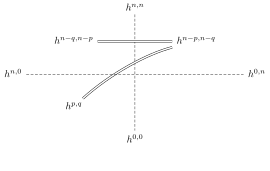This yields $$K_4$$ symmetry, and we’ll see that for a Calabi Yau there is a $$D_4$$ symmetry.

# 10 Tuesday, February 21

Last time: $$M$$ a compact manifold, $$\dim_{\mathbf{R}}M = 2n$$, there is a perfect pairing \begin{align*} H^k(M;{\mathbf{Z}})/{\operatorname{tors}}\otimes_{\mathbf{Z}}H^k(M; {\mathbf{Z}})/{\operatorname{tors}}&\to {\mathbf{Z}}\\ \alpha \otimes\beta &\mapsto \int_M \alpha\vee\beta .\end{align*} Interpret $$\alpha . \beta$$ as $$[N_1] . [N_2] = \sum_{p\in N_1 \pitchfork N_2} \pm 1$$ where the $$N_i$$ are Poincaré duals. Satisfies $$\alpha . \beta= (-1)^{n} \beta \alpha$$ for $$\alpha, \beta\in H^{n}(X; {\mathbf{Z}})/{\operatorname{tors}}$$ where $$n\mathrel{\vcenter{:}}=\dim_{\mathbf{C}}M = {1\over 2}\dim_{\mathbf{R}}M$$.

An (orthogonal) lattice is a free abelian group $$\Lambda \cong {\mathbf{Z}}^k$$ of finite rank, together with an integral symmetric bilinear form \begin{align*} \cdot: \Lambda\otimes\Lambda\to {\mathbf{Z}} .\end{align*}

• $$\Lambda$$ is symplectic if $$\cdot$$ is alternating, so $$\alpha.\beta = -\beta . \alpha$$.

• $$\Lambda$$ is unimodular if for all primitive nonzero vectors $$x\in \Lambda$$, $$\exists y\in \Lambda$$ such that $$x.y = 1$$, where $$x$$ is primitive if $$x\neq \lambda z$$ for any $$z\in \Lambda$$

• $$\Lambda$$ is nondegenerate if $$\forall x\in \Lambda$$, $$\exists y \in \Lambda$$ with $$x.y \neq 0$$.

• The Gram matrix of a basis $$\left\{{e_i}\right\}$$ for a lattice $$(\Lambda, \cdot)$$ is $$M_{ij} \mathrel{\vcenter{:}}= e_i . e_j$$. $$M$$ is symmetric for orthogonal lattices and skew-symmetric for symplectic lattices. One can write $$v.w = v^t M w$$.

• If $$\Lambda_i$$ are lattices, so is $$\bigoplus_{i} \Lambda_i$$.

The standard example: $$\Lambda \in {\mathbf{Z}}^2$$ with $${\left[ {x_1, y_1} \right]}.{\left[ {x_2, y_2} \right]} \mathrel{\vcenter{:}}= x_1 x_2 + y_1 y_2$$ is nondegenerate and unimodular. Here $${\left[ {2,2} \right]} = 2{\left[ {1,1} \right]}$$ is not primitive, but $${\left[ {2,1} \right]}$$ is primitive. Proving unimodularity: if $$\gcd(m, n) = 1$$, one just needs to solve $$mx + ny = 1$$ for $${\left[ {x,y} \right]}\in {\mathbf{Z}}^2$$. This has Gram matrix $${ \begin{bmatrix} {1} & {0} \\ {0} & {1} \end{bmatrix} }$$.

A degenerate lattice: $$({\mathbf{Z}}^2, \mathbf{x} . \mathbf{y} \mathrel{\vcenter{:}}= x_1 x_2)$$. This is symmetric, but $$\mathbf{x} . {\left[ {0, 1} \right]} = 0$$ for every $$\mathbf{x} \in \Lambda$$. The Gram matrix is $${ \begin{bmatrix} {1} & {0} \\ {0} & {0} \end{bmatrix} }$$.

A symplectic lattice: $$({\mathbf{Z}}^2, \mathbf{x} . \mathbf{y} \mathrel{\vcenter{:}}= x_1 y_2 - x_2 y_1)$$, which has Gram matrix $${ \begin{bmatrix} {0} & {1} \\ {-1} & {0} \end{bmatrix} }$$.

There is a $$2g{\hbox{-}}$$dimensional symplectic lattice for every $$g\geq 0$$ given by $${\mathbf{Z}}^{2g}_\mathrm{symp} \mathrel{\vcenter{:}}=\bigoplus_{i=1}^{g} ({\mathbf{Z}}^2, \cdot_\mathrm{symp})$$, which has Gram matrix comprised of $$g$$ diagonal blocks of $${ \begin{bmatrix} {0} & {1} \\ {-1} & {0} \end{bmatrix} }$$. This is the only unimodular symplectic lattice up to isomorphism, and is the intersection form on $$H^1(\Sigma_g; {\mathbf{Z}})$$. The vectors here can be represented by fundamental classes of 1-dimensional submanifolds, i.e. real curves: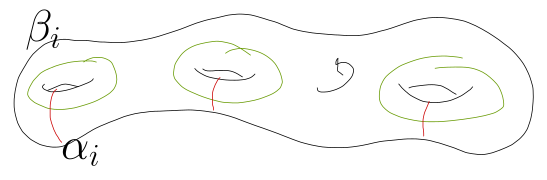More generally, if $$\dim_{\mathbf{R}}M = 4k+2$$, then $$H^{2k+1}(M; {\mathbf{Z}}) \cong {\mathbf{Z}}^{2g}_\mathrm{symp}$$ for some $$g$$.

For $$M \subseteq \Lambda$$, define $$M^\perp \mathrel{\vcenter{:}}=\left\{{x\in \Lambda{~\mathrel{\Big\vert}~}x.m =0 \, \forall m\in M}\right\}$$.

For $${\mathbf{Z}}^2_\mathrm{symp} = {\mathbf{Z}}\alpha \oplus {\mathbf{Z}}\beta$$, we have $${\mathbf{Z}}\alpha^\perp = {\mathbf{Z}}\alpha$$. For $${\mathbf{Z}}^2_\text{std}= {\mathbf{Z}}\alpha \oplus {\mathbf{Z}}\beta$$, one instead has $${\mathbf{Z}}\alpha^\perp = {\mathbf{Z}}\beta$$.

Over $${\mathbf{R}}$$, symmetric bilinear forms are classified by their signature: they can all be diagonalized to $$\operatorname{diag}(1,\cdots, 1, -1,\cdots,-1,0,\cdots, 0)$$ for some multiplicities $$n_+, n_-, n_0$$ where $$n_+ + n_- + n_0 = \operatorname{rank}_{\mathbf{Z}}\Lambda$$. Any lattice can be extended via $$\Lambda_{\mathbf{R}}\mathrel{\vcenter{:}}=\Lambda \otimes_{\mathbf{Z}}{\mathbf{R}}$$, so define $$\operatorname{sgn}\Lambda \mathrel{\vcenter{:}}=(n_+, n_-, n_0)$$.

Let $$\Lambda = {\mathbf{Z}} { \left[ {\sqrt{5}} \right] }$$ and let $$u.v = \Re(x\overline{y})$$, so \begin{align*} {\left\lVert {u} \right\rVert} = u.u = (a+b\sqrt{5})(a-b\sqrt{5}) = a^2 - 5b^2 .\end{align*} Note that $$\Lambda_{\mathbf{R}}\cong {\mathbf{R}}^{1,1}$$, which in the standard basis has Gram matrix $${ \begin{bmatrix} {1} & {0} \\ {0} & {-1} \end{bmatrix} }$$. This can be visualized as a 2-dimensional subspace of $${\mathbf{R}}^2$$ spanned by $$1, \sqrt{5}$$. Note that $${\left\lVert {\sqrt 5} \right\rVert} = -5$$.

Define the hyperbolic signature as $$(1, n)$$ for any $$n\geq 0$$. One can visualize positive/negative norm vectors using the light cone: for $${\mathbf{R}}^{1, n}$$, solving $$v.v=0$$ to get $$x_1^2 = x_2^2 + \cdots + x_{n_1}^2$$. This is a cone over $$S^1$$ at height 1 in $${\mathbf{R}}^3$$: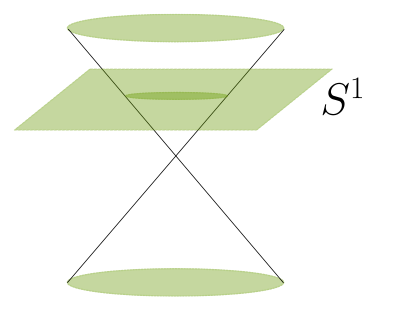Note that $$\left\{{v.v > 0}\right\}$$ has two connected components.

A hyperbolic cell/plane is the lattice $$H$$ defined as $${\mathbf{Z}}^2$$ with pairing given by the Gram matrix $${ \begin{bmatrix} {0} & {1} \\ {1} & {0} \end{bmatrix} }$$ and signature $$(1, 1)$$. This admits an orthonormal basis $${e_1 + e_2\over \sqrt 2}, {e_1 - e_2 \over \sqrt 2}$$, and $$H_{\mathbf{R}}\cong {\mathbf{R}}^{1, 1}$$.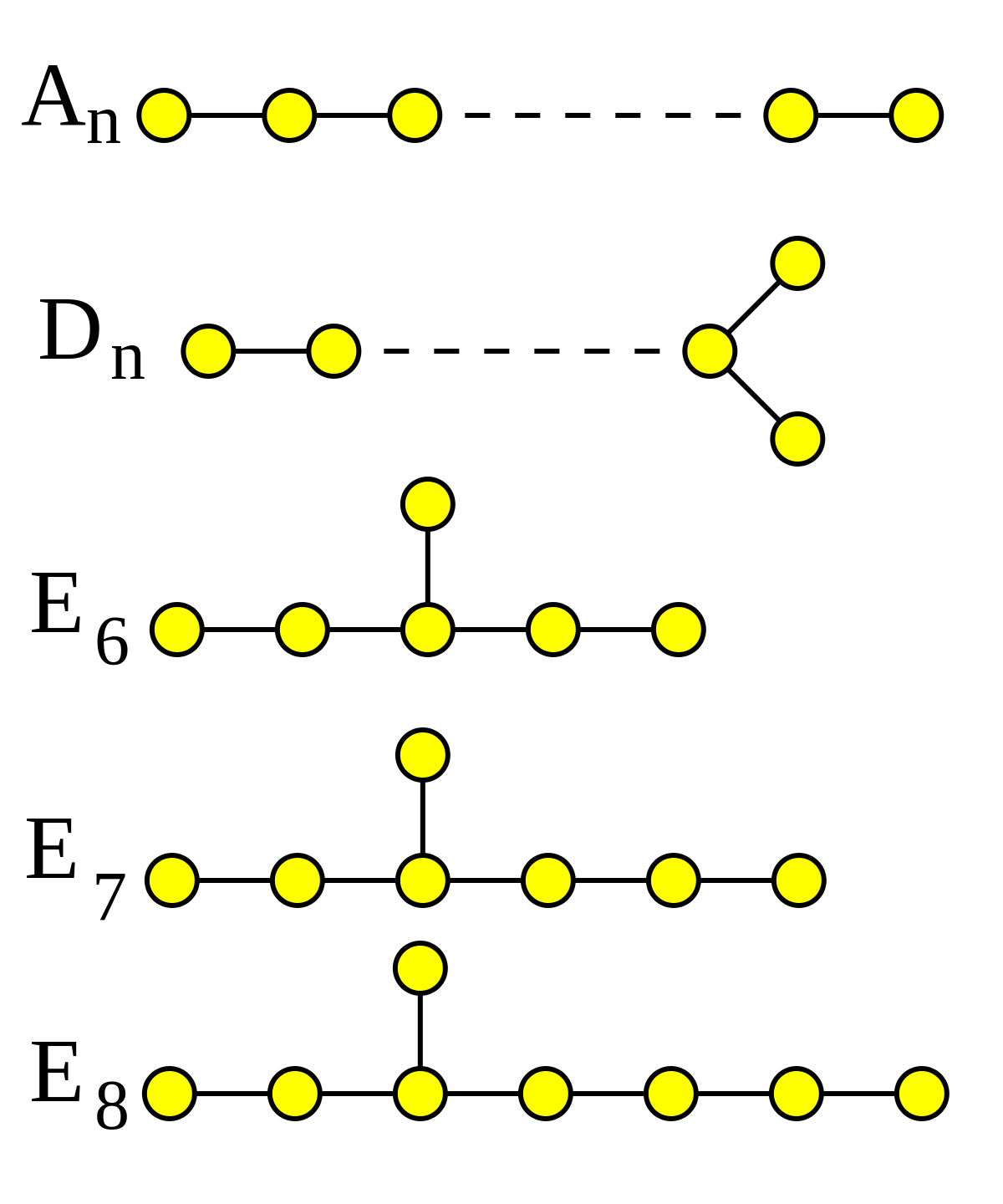One can build a root lattice out of each diagram:

• Take one basis vector $$e_i$$ for each node,
• $$e_i^2 = -2$$,
• $$e_i.e_j = 1 \iff$$ nodes $$i, j$$ are connected, and zero otherwise.

The lattices will be negative definite, i.e. of signature $$(0, n)$$ with $$n$$ the number of nodes. The only unimodular such lattice corresponds to $$E_8$$.

$$A_1$$ corresponds to the matrix $$[-2]$$, and thus $${\mathbf{Z}}$$ with bilinear form $$-2n^2$$. $$A_2$$ yields $${ \begin{bmatrix} {-2} & {1} \\ {1} & {-2} \end{bmatrix} }$$, and $$A_3$$ yields $${ \begin{bmatrix} {-2} & {1} & {0} \\ {1} & {-2} & {1} \\ {0} & {1} & {-2} \end{bmatrix} }$$.

How does one check that a lattice is unimodular?

Let $$(\Lambda, \cdot)$$ be a nondegenerate lattice, so $$\Lambda\hookrightarrow\Lambda_{\mathbf{R}}\cong {\mathbf{R}}^{a, b}$$. Define \begin{align*} \Lambda {}^{ \vee }\mathrel{\vcenter{:}}=\left\{{ y\in \Lambda_{\mathbf{R}}{~\mathrel{\Big\vert}~}x.y\in {\mathbf{Z}}\, \forall x\in \Lambda}\right\} .\end{align*}

Consider $$\Lambda \mathrel{\vcenter{:}}=\sqrt{2}{\mathbf{Z}}\hookrightarrow{\mathbf{R}}^1$$ with the standard pairing, then $$1\over \sqrt{2}\in \Lambda {}^{ \vee }$$.

One can always find a basis of $$\Lambda {}^{ \vee }$$ given by $$e_i {}^{ \vee }$$ where $$e_i {}^{ \vee }e_j = \delta_{ij}$$. Since $$e_i {}^{ \vee }M e_j = \delta_{ij}$$ for $$M$$ the Gram matrix of a form, one finds that $$e_i {}^{ \vee }$$ is the $$i$$th row of $$M^{-1}$$. Why: letting $$N$$ be the matrix with rows $$e_i {}^{ \vee }$$, one has $$NM = I$$.

$$\Lambda$$ is unimodular iff $$\Lambda {}^{ \vee }= \Lambda$$.

Note $$x^2\in {\mathbf{Z}}$$ by definition, so $$\Lambda \subseteq \Lambda {}^{ \vee }$$. If $$v\in \Lambda {}^{ \vee }\setminus\Lambda$$, then one can show that the minimal $$n$$ such that $$nv\in \Lambda$$ yields a primitive element of $$\Lambda$$. Since $$Nv.w \in n{\mathbf{Z}}$$ for all $$w$$, so can’t pair to 1.

So $$\Lambda {}^{ \vee }\mathrel{\vcenter{:}}=\bigoplus {\mathbf{Z}}e_i {}^{ \vee }\subseteq \Lambda \implies e_i {}^{ \vee }\in \Lambda \implies M^{-1}\in \operatorname{GL}_n({\mathbf{Z}})$$, and applying the same argument to duals yields $$\operatorname{det}M = \pm 1$$. In general, $$\operatorname{det}M = {\sharp}( \Lambda {}^{ \vee }/ \Lambda)^2$$ is the covolume. So

• $$\operatorname{vol}({\mathbf{R}}^n / \Lambda) = \operatorname{det}M$$
• $$\operatorname{vol}({\mathbf{R}}^n / \Lambda {}^{ \vee }) = \operatorname{det}M^{-1}$$, which is the Gram matrix of $$\Lambda {}^{ \vee }$$.
• $${\sharp}(\Lambda {}^{ \vee }/ \Lambda)^2 = \operatorname{covol}( \Lambda)^2/ \operatorname{covol}( \Lambda {}^{ \vee })^2 = \operatorname{det}(M)^2$$.

# 11 Tuesday, March 14

Today: Hirzebruch-Riemann-Roch and Chern classes. Let $$E\to X$$ be a smooth $${\mathbf{C}}{\hbox{-}}$$vector bundle, then $$\exists f: Y\to X$$ such that $$f^* E$$ splits (as a smooth $${\mathbf{C}}{\hbox{-}}$$vector bundle) into a direct sum of line bundles, i.e. $$f^* E { \, \xrightarrow{\sim}\, }\bigoplus _{i\leq r} L_i$$ where $$r\mathrel{\vcenter{:}}=\operatorname{rank}E$$. One can ensure that $$f^*: H^*(X; {\mathbf{Z}})\hookrightarrow H^*(Y; {\mathbf{Z}})$$ is injective.

This allows us to build $$c_k(E)$$ and thus $$c_k(E)$$ from the Chern roots $$x_i \mathrel{\vcenter{:}}= c_1(L_i)$$ of $$E$$. Set $$c_k(f^* E) \mathrel{\vcenter{:}}=\sigma_k(x_1,\cdots, x_r)$$, the $$k$$th elementary symmetric polynomial in the $$x_i$$. Note that $$\prod_{i\leq r}(t + x_i) = \sum_{i\leq r} s_{r-i} x^i$$ where e.g.

• $$s_1(x_1,\cdots, x_r) = \sum x_i$$
• $$s_2(x_1,\cdots, x_r) = \sum_{i < j} x_i x_j$$
• $$s_r(x_1,\cdots, x_r) = \prod x_i$$

Define \begin{align*} c(E, t) \mathrel{\vcenter{:}}=\prod_{i\leq r} (t+x_i),\qquad c(E) \mathrel{\vcenter{:}}= c(E, 1) = \sum c_i \end{align*} where $$c_i = H^{2i}(Y; {\mathbf{Z}})$$, and set $$f^*(c_k E) \mathrel{\vcenter{:}}= c_k (f^* E)$$; this uniquely defines $$c_k(E)$$.

Note on proving the splitting principle: for $$A\hookrightarrow B\twoheadrightarrow C$$ smooth vector bundles, putting a Hermitian metric on $$B$$ yields $$A^\perp$$ in $$B$$ and thus a (smooth) splitting. Set $$Y\mathrel{\vcenter{:}}=\left\{{x\in X{~\mathrel{\Big\vert}~}V_1 \subseteq V_2 \subseteq \cdots \subseteq V_r = E_x,\, \dim_{\mathbf{C}}V_i = i}\right\}$$, where $$f: Y\to X$$ by forgetting the flag. Then $$\dim Y = \dim X + \dim \operatorname{Fl}({\mathbf{C}}^r)$$, then $$f^* E$$ admits a filtration $$F^i$$ where $$F^1$$ is a line bundle. This yields SESs $$F^{i-1} \hookrightarrow F_i \twoheadrightarrow L_r$$ which split.

Define the total Chern character as $$c(E) \mathrel{\vcenter{:}}=\sum_{i\leq r} e^{x_i}$$. Note that $${\mathbf{C}}[t_1,\cdots, t_r]^{S_r} = {\mathbf{C}}[s_1, \cdots, s_r]$$, and \begin{align*} \sum_{i\leq r} \qty{\sum_{k\geq 0} { x_i^k \over k!} } &= r + (x_1+\cdots + x_r) + \qty{{x_1^2\over 2} + \cdots + {x_r^2\over 2}} + \cdots \\ &= r + c_1 + \qty{{c_1^2\over 2} - c_2} + \cdots ,\end{align*} noting that e.g. $$c_1^2 = \sum_i x_i^2 + 2 \sum_{i < j} x_i x_j$$. Define the total Todd class as \begin{align*} \operatorname{Td}(E) \mathrel{\vcenter{:}}=\prod_i {1\over 1-e^{-x_i}} = 1 + {c_1\over 2} + {c_1^2 + c_2 \over 12} + {c_1c_2 \over 24} .\end{align*} Note this is holomorphic at each $$x_i$$ by L’Hopital, and moreover symmetric, and each term is a generating function for Bernoulli numbers.

Recall that RR says that for a holomorphic line bundle of $$L$$, one can compute $$\chi(L)$$ in terms of $$\deg L = \int c_1(L)$$, a purely topological invariant. The following theorem generalizes this:

Let $$E\to X$$ be a holomorphic vector bundle over a compact complex manifold. Defining $$\chi(E) \mathrel{\vcenter{:}}=\sum (-1)^i h^0(X; E)$$, \begin{align*} \chi(E) = \int_X \mathrm{Chern}(E) \operatorname{Td}({\mathbf{T}}_X ) \end{align*} where the multiplication is in $$H^*(X;{\mathbf{Z}})$$, noting that both classes are supported in $$H^\text{even}(X;{\mathbf{Z}})$$ and the integration means taking the top degree part in $$H^{2\dim_{\mathbf{C}}X}(X; {\mathbf{Z}}) \cong {\mathbf{Z}}$$.

Recovering RR: for $$L\to X$$ a line bundle on a curve, one has $$\mathrm{Chern}(L) = e^{c_1(L)} = 1 + c_1(L)$$ and $$\operatorname{Td}(T) = {c_1(T) \over 1 - e^{-c_1(T)}} = 1 + {c_1(T)\over 2}$$. Thus \begin{align*} \chi(L) = \int_X (1 + c_1(L) )\qty{1 + {c_1(T)\over 2}} = \int_X c_1(L) + {c_1(T)\over 2} = \deg L + {1\over 2}\int_X c_1(T) .\end{align*} Note that $$c_1(T)$$ is the fundamental class of the zeros of some section of $$T$$, i.e. the number of zeros of a vector field, which by Chern-Gauss-Bonnet yields $$\int_X c_1(T) = \chi_{\mathsf{Top}}(X)$$. Thus \begin{align*} \chi(L) = \deg L + {1\over 2}\chi_{\mathsf{Top}}(X) .\end{align*} For a curve, $$\chi_{\mathsf{Top}}(X) = 2-2g$$, so $$\chi(L) = \deg L + (1-g)$$.

Let $$E\to S$$ now be a line bundle over a surface, then $$\chi(L) = h^0(L) - h^1(L) + h^2(L)$$ since $$L$$ is a coherent sheaf and $$\dim X = 2$$. HRR yields \begin{align*} \chi(L) = \int_S \qty{1 + c_1(L) + {c_2(L)\over 2!} }\qty{1 + {c_1(T)\over 2} + { c_1(T)^2 + c_2(T) \over 12}} .\end{align*} First consider the special case $$L = {\mathcal{O}}_S$$ so $$c_1(L) = 0$$ and $$\chi({\mathcal{O}}_S) = \int c_1({\mathbf{T}}_S)^2 + c_2({\mathbf{T}}_S)$$. By the splitting principle, if $${\mathbf{T}}_S = L_1 \oplus L_2$$ then $$\operatorname{det}{\mathbf{T}}_S = L_1 \otimes L_2$$, so \begin{align*} c_1({\mathbf{T}}_S) = c_1(\operatorname{det}{\mathbf{T}}_S) = c_1(L_1 \otimes L_2) = c_1(L_1) + c_1(L_2) = x_1 + x_2 .\end{align*} Note also that $$c_1(\operatorname{det}{\mathbf{T}}_S) = c_1(-K_S {}^{ \vee }) = -c_1(K_S)$$ and so $$c_1({\mathbf{T}}_S) = K_S^2$$.

For the second term, note that the top Chern class of $$E$$ is always the fundamental class of $$V(s)$$ for $$s$$ a generic smooth section of $$E$$. In particular, $$\int_S c_2({\mathbf{T}}_S) = \chi_{\mathsf{Top}}(S)$$ is the number of zeros of a smooth vector field. This yields Noether’s formula \begin{align*} \chi({\mathcal{O}}_S) = {1\over 12}\qty{K_S^2 + \chi_{\mathsf{Top}}(S)} .\end{align*}

Let $$X = {\mathbf{P}}^2$$, so $$K = {\mathcal{O}}(-3)$$ and $$\chi_{\mathsf{Top}}(X) = 3$$, so $$K^2 = (-3H)^2 = 9H^2 = 9$$ so \begin{align*} \chi({\mathcal{O}}_{{\mathbf{P}}^2}) = {1\over 12}\qty{ 9 + 3} = 1 .\end{align*} Since $$h^0({\mathcal{O}}) = 1$$ and $$h^1({\mathcal{O}}) = {1\over 2}\beta_1 = 0$$, this yields $$h^2({\mathcal{O}}) = 0$$.

RR for surfaces: \begin{align*} \chi(S, L) = \chi({\mathcal{O}}_S) + {1\over 2}(c_1({\mathbf{T}}_S) c_1(L)) + {1\over 2}c_1(L))^2 .\end{align*} Reworking this, note $$c_1({\mathbf{T}}_S) = -c_1(K_S)$$ and thus \begin{align*} \chi(S, L) = \chi({\mathcal{O}}_S) + {1\over 2}\qty{L^2 - L\cdot K_S} .\end{align*}

Computing the Hodge diamond of a K3: recall $$h^1(X) = 0$$ and $$K_X = {\mathcal{O}}_X$$.

• $$H^0: 1$$.
• $$H^1: (0, 0)$$.
• $$H^2: (1, N, 1)$$ by Serre duality for some $$N$$.
• $$H^3: (0, 0)$$ by Poincare duality.
• $$H^4: 1$$ by Poincare duality.

Computing $$N$$: $$\chi({\mathcal{O}}) = {1\over 12}(K^2 + \chi(X))$$ where $$\chi({\mathcal{O}}) = 1-0+1 = 2$$ and $$K^2 = 0$$, this yields $$\chi(X) = 24$$ and $$N = 22$$.

# 12 Thursday, March 16

For $$X$$ smooth projective, $$L\in \operatorname{Pic}(X)$$, $$V \subseteq H^0(L)$$, define $${\mathbf{P}}V$$ to be a linear system (a collection of linearly equivalent divisors) and $${\left\lvert {L} \right\rvert} \mathrel{\vcenter{:}}={\mathbf{P}}H^0(L)$$ to be a complete linear system. If $$s\in H^0(L)\setminus\left\{{0}\right\}$$, then $$V(s)\in \operatorname{Div}(X)$$ and $${\mathcal{O}}(V(s)) = L$$ (noting that all sections are linearly equivalent). Here we projectivize since $$V( \lambda s) = V(s)$$.

Let $$X = {\mathbf{P}}^1$$ and $$L = {\mathcal{O}}(1)$$, then \begin{align*} H^0(L) = \left\{{ f\in k[x,y] {~\mathrel{\Big\vert}~}f \text{ homogenous },\, \deg f = n}\right\} .\end{align*} As divisors, \begin{align*} {\mathbf{P}}H^0({\mathcal{O}}(1)) = \left\{{\sum a_i p_i {~\mathrel{\Big\vert}~}a_i > 0,\, \sum a_i = n}\right\} ,\end{align*} corresponding to the zeros and multiplicities of $$f$$.

Let $$E = {\mathbf{C}}/ \Lambda$$ be elliptic and $$L = {\mathcal{O}}(D)$$ for $$D = 3$$. Then $${\left\lvert {L} \right\rvert} = \left\{{ [p] + [q] + [r] {~\mathrel{\Big\vert}~}p+q+r =0 \operatorname{mod}\Lambda }\right\}$$. Note that $${\left\lvert {L} \right\rvert} \cong {\mathbf{P}}^2$$ since $$r$$ is determined by $$p, q$$.

Let $$C$$ be a curve with $$g\geq 2$$ and $$L = K_C = \Omega^1_C$$. Then by RR $$h^0(K_C) = g$$ and $${\left\lvert {K_C} \right\rvert} \cong {\mathbf{P}}^{g-1}$$ is called the canonical linear system.

Let $$V \leq H^0(L)$$ be a subspace and $$\left\{{s_0, \cdots, s_k}\right\}$$ be a basis. Then there is a map \begin{align*} \phi_V: X &\dashrightarrow{\mathbf{P}}^k \\ x &\mapsto [s_0(x):\cdots : s_k(x)] .\end{align*} Defining the base locus as $$\operatorname{Bs}(L) \mathrel{\vcenter{:}}=\left\{{x\in X{~\mathrel{\Big\vert}~}s_i(x) = 0 \, \forall i}\right\}$$, note $$\phi_{{\mathbf{P}}V}$$ is not well-defined for any $$x\in \operatorname{Bs}(L)$$.

For $$C$$ a curve of $$g\geq 2$$, $$\operatorname{Bs}(K_X) = \emptyset$$.

STS $$\forall p\in C$$ there is some $$s\in H^0(K_C)$$ with $$s(p) \neq 0$$. Letting $$s_p$$ be a section vanishing only at $$p$$, multiplication by $$s_p$$ induces $$H^0(K_C(-p)) \hookrightarrow H^0(K_C)$$ with image the sections of $$K_C$$ vanishing at $$p$$. Thus STS this is not surjective by showing $$h^0(K_C) > h^0(K_C(-p))$$. Apply RR and Serre duality: \begin{align*} h^0(K_C(-p)) - h^1(K_C(-p)) &= h^0(K_C(-p)) - h^0({\mathcal{O}}(p))\\ &= \deg K_C(-p) + (1-g)\\ &= (2g-3) + (1-g)\\ &= g-2 .\end{align*} Note that if $$s\in H^0({\mathcal{O}}(p))$$ then $$V(s) = [q]$$ must be a single point, but if $$p\neq q$$ then $$[p]-[q] = 0$$ and $$\exists f: C\to {\mathbf{P}}^1$$ with $$f^{-1}(0) = p$$ and $$f^{-1}(\infty) = q$$ with $$\deg f = 1$$, forcing $$C \cong {\mathbf{P}}^1$$ and contradicting $$g \geq 2$$. So $$p=q$$, and $$h^0({\mathcal{O}}(p)) = 1$$, and thus \begin{align*} h^0(K_C(-p)) - 1 = g-2 \implies h^0(K_C(-p)) = g - 1 < g = h^0(K_C) .\end{align*}

Show that if $$C$$ is not hyperelliptic ($$\exists f: C\to {\mathbf{P}}^1$$ 2-to-1) then $$\forall p,q\in C$$ one can find $$s \in H^0(K_C)$$ with $$s(p) = 0, s(q)\neq 0$$, so they are separated by linear forms on $${\mathbf{P}}^{g-1}$$. This yields an actual morphism $$\phi_{{\left\lvert {K_C} \right\rvert}}: C\to {\mathbf{P}}^{g-1}$$ where $$p, q$$ are not mapped to the same point. This is the canonical embedding of a curve, which only works when $$g\geq 3$$ and $$C$$ is non-hyperelliptic. If $$g = 2$$ or $$C$$ is hyperelliptic, $$3K_C$$ yields an (tricanonical) embedding.

If $$C$$ is not hyperelliptic and $$g = 3$$, then $$C\hookrightarrow{\mathbf{P}}^2$$ by the canonical embedding. This yields an element in $$\operatorname{Div}({\mathbf{P}}^2)$$, which is a smooth quartic.

\begin{align*} K_{{\mathbf{P}}^n} = {\mathcal{O}}(-n-1) .\end{align*}

Take coordinates $$[x_0:\cdots : x_n]$$ and take $$\omega \mathrel{\vcenter{:}}={\,dx_1\over x_1} \wedge \cdots \wedge {\,dx_n\over x_n}$$, noting that this omits $$x_0$$. This has poles along each $$V(x_i)$$ for $$i\neq 0$$ and in fact a pole at $$x_0$$, since these are $$n+1$$ distinct spaces. E.g. for $$n=1$$, since $$x_1 = x_0^{-1}$$ we have $${ d(x^{-1})\over x^{-1}} = - {\,dx_1\over x_1}$$.

If $$X$$ is smooth and $$D\in \operatorname{Div}(X)$$ then \begin{align*} K_D = { \left.{{\qty{K_X \otimes{\mathcal{O}}(D)}}} \right|_{{D}} } .\end{align*}

Omitted, take residues.

Applying this to the previous curve situation: note $$\deg K_C = 2g-2 = 4$$, which counts $$\phi_{{\left\lvert {K_C} \right\rvert}}(C) \cap V(x_0)$$ and yields a quartic.

For $$C$$ a degree $$d$$ curve in $${\mathbf{P}}^2$$, \begin{align*} g = {d-1\choose 2} .\end{align*}

Take degrees in the adjunction formula and apply Bezout’s theorem: \begin{align*} K_{C} = K_{{\mathbf{P}}^2} + C \mathrel{\Big|}_C \implies 2g(C) - 2 = = \deg(-3H + dH)\mathrel{\Big|}_C = d (d-3) .\end{align*} More generally if $$C \subseteq S$$ a curve in a surface, $$\deg L \mathrel{\Big|}_C = c_1(L) . [C]$$ is an intersection number. Expanding this yields $$g = {d^2 - 3d +2 \over 2} = {d-1\choose 2}$$.

\begin{align*} \kappa(X) \mathrel{\vcenter{:}}=\max_{n > 0} \left\{{\dim \operatorname{im}\phi_{nK_X} }\right\} \end{align*} where $$\dim \emptyset \mathrel{\vcenter{:}}=-\infty$$ and $$\kappa(X) \in \left\{{-\infty,0,\cdots, \dim X}\right\}$$.

Note that since $$3K_C$$ yields an embedding for a curve with $$g\geq 2$$, $$\kappa(C) = 1$$. Also note that $$\kappa(X) = -\infty \iff h^0(nK_X) = 0$$ for all $$n$$. For curves:

$$g(C)$$ $$\kappa(C)$$
$$0$$ $$-\infty$$
$$1$$ $$0$$
$$2$$ $$1$$
$$3$$ $$1$$
$$4$$ $$\vdots$$

Here we’ve used that $$K_{{\mathbf{P}}^1} = {\mathcal{O}}(-2)$$ has no sections and $$K_E = {\mathcal{O}}_E$$ is trivial. Fanos are $$\kappa = -\infty$$ and general type ($$\kappa(X) = \dim X$$) are $$g\geq 2$$.

For smooth projective surfaces: $$\kappa(S) \in \left\{{ -\infty,0,1,2 }\right\}$$. See Beauville for the Enriques-Kodaira classification due to the Italian school:

• $$\kappa(S) = -\infty$$: ruled and rational.
• $$\kappa(S) = 0$$: K3, Abelian, Enriques, Bi-elliptic.
• $$\kappa(S) = 1$$: Elliptic.
• $$\kappa(S) = 2$$: General type.

$$S$$ is ruled if $$\exists \pi: S\to C$$ with generic fiber $$\cong {\mathbf{P}}^1$$: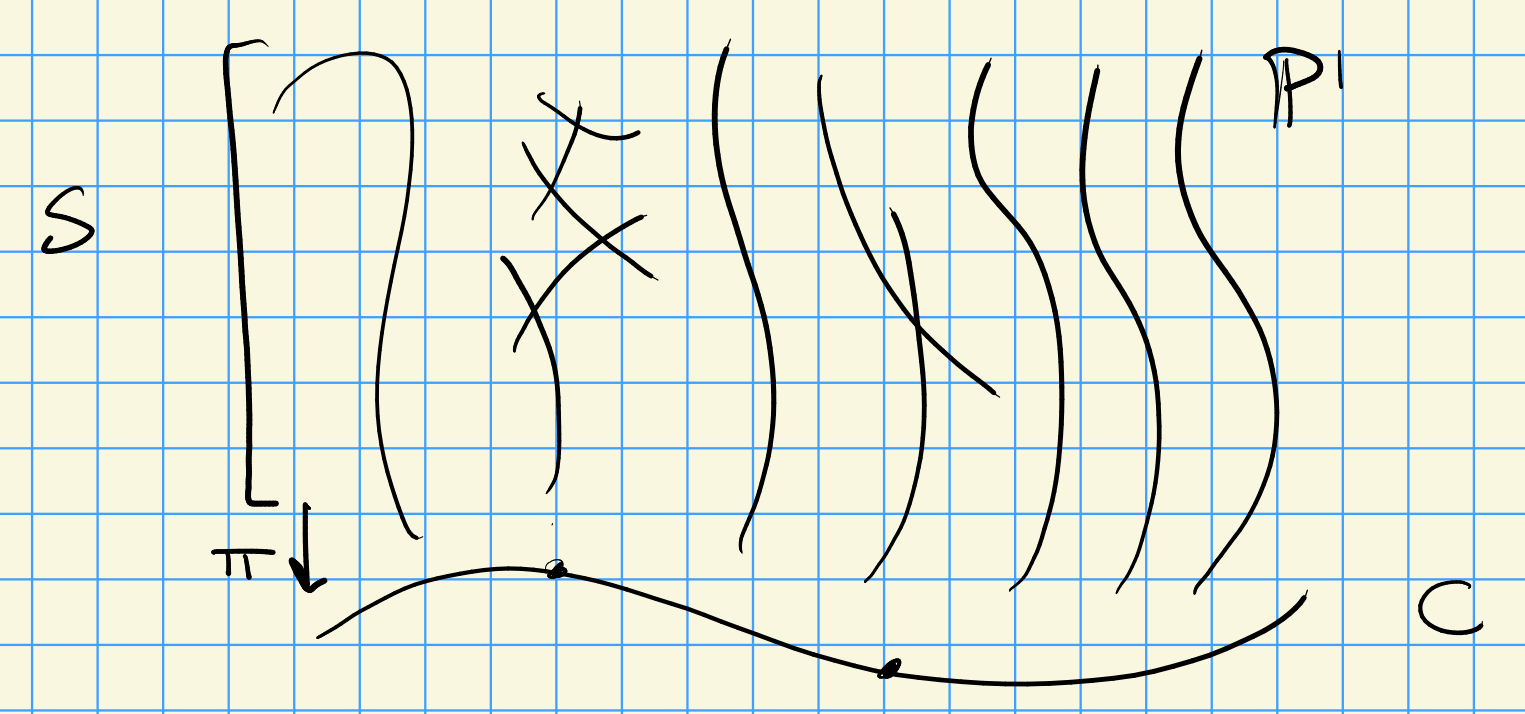$$S$$ is rational if $$S\overset{\sim}{\dashrightarrow}{\mathbf{P}}^2$$.